# JP2004015106A - Image processor and image processing method, program and program recording medium, and data structure and data recording medium - Google Patents

Image processor and image processing method, program and program recording medium, and data structure and data recording medium Download PDF

## Info

Publication number
JP2004015106A
JP2004015106A JP2002161838A JP2002161838A JP2004015106A JP 2004015106 A JP2004015106 A JP 2004015106A JP 2002161838 A JP2002161838 A JP 2002161838A JP 2002161838 A JP2002161838 A JP 2002161838A JP 2004015106 A JP2004015106 A JP 2004015106A
Authority
JP
Japan
Prior art keywords
image data
pixel
pixel value
image
point
Prior art date
Legal status (The legal status is an assumption and is not a legal conclusion. Google has not performed a legal analysis and makes no representation as to the accuracy of the status listed.)
Granted
Application number
JP2002161838A
Other languages
Japanese (ja)
Other versions
JP4211292B2 (en
JP2004015106A5 (en
Inventor
Tsugihiko Haga
Tetsujiro Kondo
Kenji Tanaka

Original Assignee
Sony Corp
ソニー株式会社
Priority date (The priority date is an assumption and is not a legal conclusion. Google has not performed a legal analysis and makes no representation as to the accuracy of the date listed.)
Filing date
Publication date
Application filed by Sony Corp, ソニー株式会社 filed Critical Sony Corp
Priority to JP2002161838A priority Critical patent/JP4211292B2/en
Publication of JP2004015106A publication Critical patent/JP2004015106A/en
Publication of JP2004015106A5 publication Critical patent/JP2004015106A5/ja
Application granted granted Critical
Publication of JP4211292B2 publication Critical patent/JP4211292B2/en
Application status is Expired - Fee Related legal-status Critical
Anticipated expiration legal-status Critical

• 239000002609 media Substances 0 title 2
• 238000003672 processing method Methods 0 title 1
• 230000000875 corresponding Effects 0 abstract 3
• 238000003384 imaging method Methods 0 abstract 1

## Images

••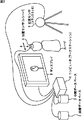•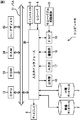•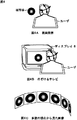•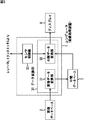•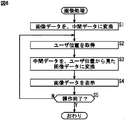•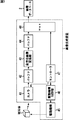•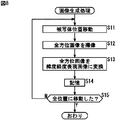•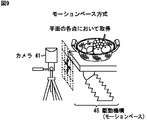•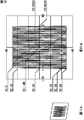•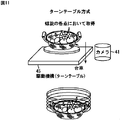•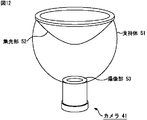•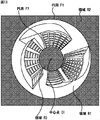•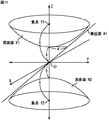•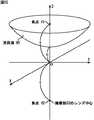•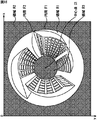•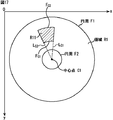•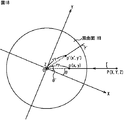•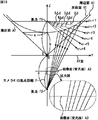•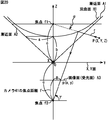•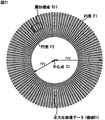•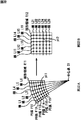•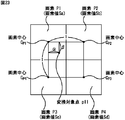•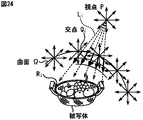•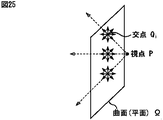•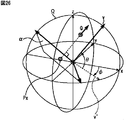•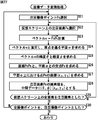•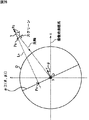•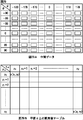••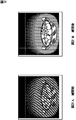•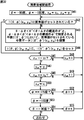•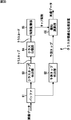•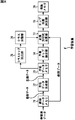•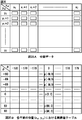•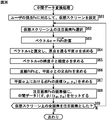•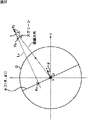•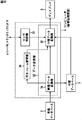•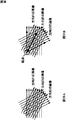•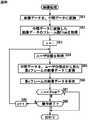•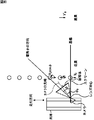•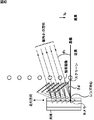•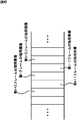•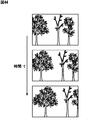•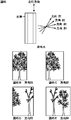•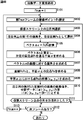•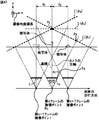•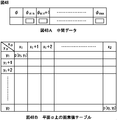•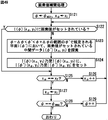•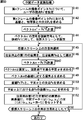••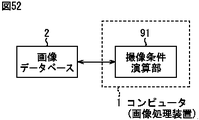•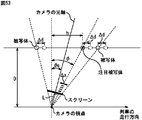•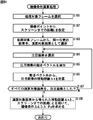## Classifications

• GPHYSICS
• G06COMPUTING; CALCULATING; COUNTING
• G06TIMAGE DATA PROCESSING OR GENERATION, IN GENERAL
• G06T3/00Geometric image transformation in the plane of the image
• G06T3/0062Panospheric to cylindrical image transformation
• GPHYSICS
• G06COMPUTING; CALCULATING; COUNTING
• G06TIMAGE DATA PROCESSING OR GENERATION, IN GENERAL
• G06T15/003D [Three Dimensional] image rendering
• G06T15/06Ray-tracing
• GPHYSICS
• G06COMPUTING; CALCULATING; COUNTING
• G06TIMAGE DATA PROCESSING OR GENERATION, IN GENERAL
• G06T15/003D [Three Dimensional] image rendering
• G06T15/10Geometric effects
• GPHYSICS
• G06COMPUTING; CALCULATING; COUNTING
• G06TIMAGE DATA PROCESSING OR GENERATION, IN GENERAL
• G06T15/003D [Three Dimensional] image rendering
• G06T15/50Lighting effects
• HELECTRICITY
• H04ELECTRIC COMMUNICATION TECHNIQUE
• H04NPICTORIAL COMMUNICATION, e.g. TELEVISION
• H04N5/00Details of television systems
• H04N5/222Studio circuitry; Studio devices; Studio equipment ; Cameras comprising an electronic image sensor, e.g. digital cameras, video cameras, TV cameras, video cameras, camcorders, webcams, camera modules for embedding in other devices, e.g. mobile phones, computers or vehicles
• H04N5/262Studio circuits, e.g. for mixing, switching-over, change of character of image, other special effects ; Cameras specially adapted for the electronic generation of special effects
• HELECTRICITY
• H04ELECTRIC COMMUNICATION TECHNIQUE
• H04NPICTORIAL COMMUNICATION, e.g. TELEVISION
• H04N7/00Television systems
• H04N7/18Closed circuit television systems, i.e. systems in which the signal is not broadcast

## Abstract

An image is easily changed according to a user's viewpoint.
An original image data of an image of a subject is stored from an arbitrary point on a curved surface Ω as a viewpoint. Focused on straight line L i connecting the R i point on the viewpoint P and the object, to represent the intersection of the straight line L i and the curved surface Ω and Q i, when viewed object from the viewpoint P, on the subject pixel values corresponding to the light beam directed to the viewpoint P from point R i coincides with the pixel value corresponding to a light beam toward a point Q i on the curved surface Ω terms R i on the object, the point Q i from the point R i The pixel value corresponding to the ray going to exists in the original image data. That is, the pixel value when viewing the point R i on the subject from the viewpoint P can be obtained from the original image data captured using the point Q i on the curved surface Ω as the viewpoint.
[Selection] Fig. 24

## Description


TECHNICAL FIELD OF THE INVENTION
The present invention relates to an image processing apparatus and an image processing method, a program and a program recording medium, and a data structure and a data recording medium, for example, an image processing apparatus and an image capable of displaying an image corresponding to a user's viewpoint. The present invention relates to a processing method, a program, a program recording medium, and a data structure and a data recording medium.

[Prior art]
In a conventional display using, for example, a CRT (Cathod Ray Tube), a liquid crystal panel, a projector, or the like, for example, an image captured by a video camera (hereinafter, also simply referred to as a camera as appropriate) is displayed. The image was only a view of the camera position as a viewpoint, as shown in FIG.

Therefore, even if the user moves the head and changes the viewpoint when viewing the image displayed on the display, for example, the image displayed on the display is displayed at the center of the lens of the camera (hereinafter, the viewpoint of the camera as appropriate). ), And the image displayed on the display did not change according to a change in the user's viewpoint.

[Problems to be solved by the invention]
Therefore, as a method of displaying an image in which the image displayed on the display changes according to the viewpoint of the user, for example, there is a method called zebra @ imaging. Here, zebra @ imaging is described in, for example, http: //www.zebra@imaging. zebraimaging. com /.

However, zebra @ imaging is a method using holography, and since a displayed image is a hologram, it takes a large amount of calculation and time to create the hologram.

Further, as another display method of an image in which an image displayed on the display changes according to the user's viewpoint, for example, IPT (Immersive Projection Technology) represented by the CAVE (Cave) method of the University of Illinois, etc. ) (Immersive projection technology) or VR (Virtual Reality) system using IPD (Immersive Projection Display) (immersive projection display). Here, as for the CAVE method, for example, http://www.web.org/electronic/visualization/laboratory. evl. uic. edu / evl / vr /.

However, in such a VR system, a target image is CG (Computer @ Graphics), and it is difficult to target a so-called real image picked up by a video camera.

The present invention has been made in view of such a situation, and it is an object of the present invention to be able to easily change even a real photographed image according to a user's viewpoint.

[Means for Solving the Problems]
A first image processing apparatus according to the present invention converts a first image data into a viewpoint of a user by using a trajectory of a light ray when the first image data is captured and light ray information which is a pixel value corresponding to the light ray. A conversion unit that converts the image data into second image data that can be seen from the outside.

A first image processing method according to the present invention uses a trajectory of a light ray when the first image data is captured and light ray information that is a pixel value corresponding to the light ray to convert the first image data into a viewpoint of a user. A conversion step of converting the image data into second image data that can be seen from the camera.

A first program according to the present invention allows a user to view first image data from a user's viewpoint using a trajectory of a light beam when the first image data is captured and light beam information that is a pixel value corresponding to the light beam. A conversion step of converting the image data into second image data.

The first program recording medium of the present invention uses the trajectory of a light ray at the time of capturing the first image data and light ray information that is a pixel value corresponding to the light ray to convert the first image data into a user's viewpoint. A program having a conversion step of converting the image data into second image data that can be seen from the computer.

The second image processing apparatus of the present invention converts the image data into a pixel value at an intersection of a light ray incident on the predetermined pixel and a plane perpendicular to the light ray and passing through a predetermined point. It is characterized by comprising a conversion means for converting into intermediate data which is image data as a value.

The second image processing method according to the present invention is a method of converting image data into a pixel value at an intersection of a light ray incident on a predetermined pixel and a plane perpendicular to the light ray and passing through a predetermined point. A conversion step of converting the data into intermediate data which is image data having a value.

A second program according to the present invention is a program for converting image data into a pixel value at an intersection of a light ray incident on a predetermined pixel and a plane perpendicular to the light ray and passing through a predetermined point, as a pixel value of the predetermined pixel. A conversion step of converting the converted image data into intermediate data.

The second program recording medium according to the present invention is a program recording medium which converts a pixel value at an intersection of a light ray incident on a predetermined pixel and a plane perpendicular to the light ray and passing through a predetermined point into a pixel of the predetermined pixel. A program having a conversion step of converting into intermediate data which is image data having a value is recorded.

According to the data structure of the present invention, an intersection between a light ray incident on a predetermined pixel constituting the original image data and a plane perpendicular to the light ray and passing through a predetermined point is defined as a pixel, and a pixel value of the pixel has a predetermined value. Is characterized by the pixel value of the pixel.

The data recording medium of the present invention is a pixel, the intersection of a light ray incident on a predetermined pixel constituting the original image data, and a plane perpendicular to the light ray and passing through a predetermined point, the pixel value of the pixel, It is characterized in that image data having a pixel value of a predetermined pixel is recorded.

In a first image processing apparatus, an image processing method, a program, and a program recording medium according to the present invention, a trajectory of a light beam when capturing first image data and light beam information that is a pixel value corresponding to the light beam are used. Thus, the first image data is converted into second image data that can be viewed from the user's viewpoint.

In the second image processing apparatus and image processing method, and the program and the program recording medium according to the present invention, the image data includes a light ray incident on the predetermined pixel and a plane passing through a predetermined point perpendicular to the light ray. Is converted to intermediate data which is image data in which the pixel value at the intersection of is a pixel value of a predetermined pixel.

In the data structure and the data recording medium of the present invention, the intersection of a light ray incident on a predetermined pixel constituting the original image data and a plane perpendicular to the light ray and passing through a predetermined point is defined as a pixel, and the pixel Is the pixel value of a predetermined pixel.

Here, the image data may be a still image or a moving image. Further, the image data may be CG, for example, if it is real image data.

BEST MODE FOR CARRYING OUT THE INVENTION
FIG. 2 shows a configuration example of an embodiment of an image display device to which the present invention is applied.

The computer 1 is, for example, a workstation, converts original image data stored in the image database 2 into intermediate data described later, and stores the intermediate data in the intermediate database 3. In addition, the computer 1 controls the receiver 4 and the transceiver 5 as position sensors, and detects the position (and, if necessary, the posture) of the user based on the output of the receiver 4. Further, the computer 1 sets the position of the user as the position of the viewpoint of the user, and sets the intermediate data stored in the intermediate database 3 to image data viewed from the viewpoint of the user (hereinafter referred to as presentation image data as appropriate). ). Then, the computer 1 supplies the display image data to the display 6 to display the display image data.

The image database 2 stores original image data as image data of a real image captured by an imaging device such as a camera, as described later. The intermediate database 3 stores intermediate data supplied from the computer 1 and converted from the original image data.

The receiver 4 is mounted on a user, detects a magnetic field generated by the transmitter 5, and supplies the intensity of the magnetic field to the computer 1. The transmitter 5 generates a magnetic field under the control of the computer 1. That is, in the embodiment shown in FIG. 2, the receiver 4 and the transmitter 5 constitute a magnetic field sensor as a position sensor, and the receiver 4 measures the intensity of the magnetic field generated by the transmitter to determine the position of the receiver 4. Thus, the position of the user is detected.

Here, the receiver 4 is mounted, for example, on the head of the user. When the user wears a tie pin or wears glasses, for example, the receiver 4 can be mounted on the tie pin or the frame of the glasses.

In addition, as a magnetic field sensor as a position sensor, for example, a three-dimensional position sensor manufactured by Pohemus can be used.

Further, as the position sensor, for example, a mechanical link type position measuring means (for example, a three-dimensional digitizer) can be employed in addition to the magnetic field sensor. Further, as the position sensor, for example, a means such as a joystick or a trackball that can input a change in the position of the user can be employed. That is, here, the position sensor does not need to be a unit for detecting the actual position of the user, but may be a unit capable of inputting the position of the virtual user.

The display 6 is composed of, for example, a CRT or a liquid crystal panel, and displays presentation image data supplied from the computer 1. As the display 6, in addition to the CRT and the liquid crystal panel, for example, a projector, an HMD (Head Mount Display), or other means capable of displaying an image can be adopted.

Next, FIG. 3 shows an example of a hardware configuration of the computer 1 of FIG.

The computer 1 has installed therein a program for executing a series of processes described later.

The program can be recorded in advance on a hard disk 15 or a ROM 13 as a recording medium built in the computer 1.

Alternatively, the program may be temporarily or temporarily stored on a removable recording medium 21 such as a flexible disk, a CD-ROM (Compact Disc Only), an MO (Magneto Optical) disc, a DVD (Digital Versatile Disc), a magnetic disc, and a semiconductor memory. It can be stored (recorded) permanently. Such a removable recording medium 21 can be provided as so-called package software.

The program is installed in the computer 1 from the removable recording medium 21 as described above, and is wirelessly transferred from the download site to the computer 1 via an artificial satellite for digital satellite broadcasting, or a LAN (Local Area Network). ), The program can be transferred to the computer 1 via a network such as the Internet by wire, and the computer 1 can receive the transferred program by the communication unit 18 and install the program on the built-in hard disk 15.

The computer 1 has a built-in CPU (Central Processing Unit) 12. An input / output interface 20 is connected to the CPU 12 via the bus 11. The CPU 12 operates the input unit 17 including a keyboard, a mouse, and a microphone by the user via the input / output interface 20. When a command is input by performing the above, the program stored in the ROM (Read Only Memory) 13 is executed according to the command. Alternatively, the CPU 12 may execute a program stored in the hard disk 15, a program transferred from a satellite or a network, received by the communication unit 18 and installed in the hard disk 15, or a removable recording medium 21 mounted in the drive 19. The program that has been read and installed on the hard disk 15 is loaded into a RAM (Random Access Memory) 14 and executed. Accordingly, the CPU 12 performs a process according to a flowchart described later or a process performed by a configuration of a block diagram described later. Then, the CPU 12 outputs the processing result as necessary, for example, from the output unit 16 formed of a speaker or the like via the input / output interface 20 or transmits the processing result from the communication unit 18. Record.

In the embodiment shown in FIG. 3, the image database 2, the intermediate database 3, the receiver 4, the transmitter 5, and the display 6 are connected to the input / output interface 20. Then, the CPU 12 reads out the original image data stored in the image database 2 via the input / output interface 20 and converts it into intermediate data. Further, the CPU 12 stores the intermediate data in the intermediate database 3 via the input / output interface 20. Further, the CPU 12 controls the transmitter 5 via the input / output interface 20, receives the output of the receiver 4, and detects the position of the user. Further, the CPU 12 sets the detected position of the user as the viewpoint of the user, and converts the intermediate data stored in the intermediate database 3 into image data for presentation in accordance with the viewpoint of the user. Further, the CPU 12 supplies the display image data to the display 6 via the input / output interface 20 to display the display image data.

Here, in the present specification, processing steps for describing a program for causing the computer 1 to perform various processes do not necessarily need to be processed in chronological order in the order described as a flowchart described later, and may be performed in parallel. Alternatively, it also includes processing executed individually (for example, parallel processing or processing by an object).

Further, the program may be processed by one computer, or may be processed in a distributed manner by a plurality of computers. Further, the program may be transferred to a remote computer and executed.

In the image display apparatus of FIG. 2 configured based on the computer 1 of FIG. 3 as described above, for example, as shown in FIG. The image data is displayed.

That is, when the user observes a certain subject in the real world, when the user moves the viewpoint, the user can observe the subject viewed from the viewpoint after the movement. Specifically, in the real world, as the user moves his / her viewpoint, the visible part and the invisible part of the subject change.

In the image display device of FIG. 2 as well, as shown in FIG. 4B, when the user moves the viewpoint, the presentation image data displayed on the display 6 according to the viewpoint changes the visual image of the user in the real world. Is displayed on the screen. That is, in the image display device of FIG. 2, as shown in FIG. 4C, when the user moves the viewpoint, presentation image data when the subject is viewed from the viewpoint is displayed.

Therefore, in the image display device of FIG. 2, when the user moves the viewpoint, for example, the presentation image data in which the part of the subject that cannot be seen from the viewpoint before the movement is visible is displayed. In other words, when the user moves the viewpoint so as to look into the subject displayed in the image data for presentation on the display 6, in the real world, the same as when the subject is viewed from the viewpoint after the movement is visually recognized. The presentation image data, that is, the presentation image data in which the portion of the subject that the user has looked into is visible. From this, the image display device of FIG. 2 can be said to be a so-called television (Television).

Next, FIG. 5 shows an example of a functional configuration of an image processing apparatus realized by the computer 1 of FIG. 3 executing a program.

An image processing apparatus realized by (the CPU 12 of) the computer 1 executing a program includes a data conversion unit 30 and a sensor control unit 33.

The data conversion unit 30 is composed of an image data conversion unit 31 and an intermediate data conversion unit 32, and is a trajectory of a light ray when capturing the original image data stored in the image database 2 and a light ray that is a pixel value corresponding to the light ray. Using the information, the original image data is converted into presentation image data supplied from the sensor control unit 33 and viewed from the user's viewpoint, and supplied to the display 6 for display.

That is, the data conversion unit 31 reads the original image data from the image database 2 and geometrically converts the data into intermediate data. Further, the data conversion unit 31 supplies the intermediate data to the intermediate database 3 and stores it.

The intermediate data conversion unit 32 converts the intermediate data into image data for presentation based on the user's viewpoint supplied from the sensor control unit 33, that is, reconstructs image data viewed from the user's viewpoint, 6 to be displayed.

The sensor control unit 33 detects the user's viewpoint by controlling the receiver 4 and the transmitter 5 as the position sensors, and supplies the viewpoint to the intermediate data conversion unit 32. That is, the sensor control unit 33 generates a magnetic field by controlling the transmitter 5. Further, the sensor control unit 33 detects the position of the user based on the detection result of the magnetic field generated by the transmitter 5 supplied from the receiver 4, sets the position as the user's viewpoint, and converts the intermediate data. To the unit 32.

Next, image processing by the image processing apparatus (computer) 1 in FIG. 5 will be described with reference to the flowchart in FIG.

First, in step S1, the data conversion unit 31 reads the original image data from the image database 2 and converts it into intermediate data. Further, in step S1, the data conversion unit 31 supplies the intermediate data to the intermediate database 3 for storage, and proceeds to step S2.

In step S2, the sensor control unit 33 detects the position of the user from the output of the receiver 4, sets the position of the user to the user's viewpoint, and supplies the user's viewpoint to the intermediate data conversion unit 32.

Then, the process proceeds to step S3, where the intermediate data conversion unit 32 converts the intermediate data into image data for presentation based on the user's viewpoint supplied from the sensor control unit 33, and then proceeds to step S4.

In step S4, the intermediate data conversion unit 32 supplies the image data for presentation to the display 6 for display, and proceeds to step S5.

In step S5, for example, the CPU 12 (FIG. 3) determines whether or not the user has operated the input unit 17 (FIG. 3) so as to end the image processing. If it is determined in step S5 that the input unit 17 has not been operated so as to end the image processing, the process returns to step S2, and the same processing is repeated.

If it is determined in step S5 that the input unit 17 has been operated so as to end the image processing, the processing ends.

If the image processing of FIG. 6 has been executed in the past, and the intermediate data obtained by converting the original image data stored in the image database 2 is already stored in the intermediate database 3, The processing of S1 can be skipped.

Next, FIG. 7 illustrates a configuration example of an image generating apparatus that generates (imaging) original image data to be stored in the image database 2.

In the embodiment of FIG. 7, the camera 41 is configured by, for example, a so-called omnidirectional camera, and captures omnidirectional image data (omnidirectional image data) including a subject under the control of the controller 47, and stores the image data in the buffer 42. Supply.

The buffer 42 temporarily stores the omnidirectional image data supplied from the camera 41.

The omnidirectional image conversion unit 43 reads the omnidirectional image data stored in the buffer 42, converts the read omnidirectional image data into original image data expressed in latitude and longitude, and supplies the original image data to the buffer 44.

The buffer 44 temporarily stores original image data supplied from the omnidirectional image converter 43.

The drive mechanism 45 moves the subject to be imaged by the camera 41 under the control of the drive mechanism control unit 46. Here, as the subject is moved by the drive mechanism 45, the viewpoint (optical center) of the camera 41 with respect to the subject is relatively changed to a plurality of positions.

The drive mechanism control unit 46 drives the drive mechanism under the control of the controller 47.

The controller 47 controls the imaging timing of the camera 41, the driving speed and the driving direction of the driving mechanism 45 by the driving mechanism control unit 46, and the like. Further, the controller 47 transmits a mux (multiplexer) 48 with information indicating an imaging timing (imaging time) of the camera 41 and information indicating the position of the subject moved by the driving mechanism 45 (including the posture of the subject as necessary). To supply.

The multiplexer 48 adds information output from the controller 47 for each frame of the original image data stored in the buffer 44 to the frame, and supplies the frame to the image database 2 for storage.

Next, an image generation process by the image generation device of FIG. 7 will be described with reference to a flowchart of FIG.

First, the controller 47 controls the camera 41 and the drive mechanism control unit 46 to move the subject to a predetermined position and perform imaging of the subject, and transmits information indicating the predetermined position and the imaging timing of the subject. , Mux 48.

Here, for example, the controller 47 previously sets a three-dimensional coordinate system (hereinafter, appropriately referred to as an imaging coordinate system) in the real world, and converts the coordinates of the subject in the imaging coordinate system into information representing the position of the subject. (Hereinafter referred to as position information as appropriate). Further, the controller 47 sets a certain posture of the subject (for example, the posture of the subject at the start of imaging) as a reference posture, and outputs an angle representing a change of the subject from the reference posture in the position information. Further, the controller 47 outputs the coordinates indicating the position of the camera 41 (for example, the position of the lens center) in the coordinate system at the time of imaging and the information indicating the optical axis direction (the direction of the line of sight) in the position information. .

When the subject is moved by the motion base method described in FIG. 9 described later or the turntable method described in FIG. 11, the position of the lens center (optical center) and the optical axis direction of the camera 41 are changed. Instead, the position of the lens center of the camera 41 and the optical axis direction are set in the controller 47 in advance.

In addition, the above-described coordinates and posture of the subject are actually grasped from the state of the drive mechanism 45 that moves the subject. Here, the state of the drive mechanism 45 is recognized by a controller 47 that controls the drive mechanism 45 via a drive mechanism control unit 46.

In step S11, the drive mechanism control unit 46 changes the position of the subject by controlling the drive mechanism 45 in accordance with the control of the controller 47, and proceeds to step S12. In step S12, the camera 41 captures an image of the subject under the control of the controller 47, and supplies the resulting omnidirectional image data to the buffer 42 for storage.

Then, the process proceeds to step S13, where the omnidirectional image conversion unit 43 reads the omnidirectional image data stored in the buffer 42, converts the omnidirectional image data into original image data expressed in latitude and longitude, and supplies the original image data to the buffer 44. Thereafter, the process proceeds to step S14, where the mux 48 adds information supplied from the controller 47 to the frame of the original image data stored in the buffer 44, and supplies the frame to the image database 2 for storage.

After the process in step S14, the process proceeds to step S15, in which the controller 47 determines whether or not the subject has been moved to all preset positions (further, if necessary, whether or not all preset postures have been taken). ), If it is determined that the object has not yet been moved to all positions, the camera 41 and the drive mechanism control unit 46 are controlled so that the subject is moved to a position that has not been moved yet and an image thereof is taken. At the same time, information indicating the moving position of the subject and the imaging timing of the subject and the like are supplied to the mux 48. Then, the process returns to step S11, and thereafter, the same processing is repeated.

If it is determined in step S15 that the subject has been moved to all the preset positions, that is, if the subject has been imaged at all the preset positions, the process ends.

Next, a description will be given of a subject moving method for moving the subject in the image generating apparatus of FIG.

In the image generating apparatus of FIG. 7, the imaging is performed while moving the subject. In this case, it is necessary to control the position of the subject with high accuracy. Examples of such a method of moving the subject include a motion-based method and a turntable method.

In the motion base method, as shown in FIG. 9, a motion base is used as the drive mechanism 45. Then, the subject is placed on the motion base as the driving mechanism 45, and the motion base is moved up and down and left and right (toward the camera 41), for example, so that the subject moves in parallel.

That is, in the motion base method, the motion base as the drive mechanism 45 is gradually moved from left to right while moving vertically as shown in FIG. 10A, for example. Here, FIG. 10B shows an actual motion-based trajectory. In FIG. 10B, the numbers displayed after TC: together with the motion-based trajectory represent the time code of the image captured by the camera 41. Therefore, in FIG. 10B, since the motion-based trajectory is associated with the time code, it is possible to recognize the correspondence between the position (posture) of the subject and the frame of the image data of the subject at that position. Can be.

According to the motion-based method of FIG. 9, when an image of a subject is taken as an open curved surface that can be assumed between the subject and the camera 41, for example, when a plurality of points on a plane are taken as viewpoints, Ray information, which is a pixel value corresponding to the trajectory and the ray, can be obtained. The pixel value corresponding to the light beam from the subject can be obtained from the image data output by the camera 41, and the trajectory of the light beam from the subject can be obtained from the camera 41 and the position of the subject.

In the turntable method, as shown in FIG. 11, a turntable (cart) is used as the drive mechanism 45. Then, the subject is placed on a turntable as the drive mechanism 45, and the turntable is rotated at a constant angular velocity while moving upward or downward at a very low speed.

According to the turntable method of FIG. 11, it is possible to obtain light beam information when an image of a subject is taken using, for example, a plurality of points on the side surface of a cylinder as an open curved surface surrounding the subject.

Note that, in the image generation device of FIG. 7, by moving a subject, image data obtained by viewing the subject from each of a plurality of viewpoints is taken. However, instead of moving the subject, the camera 41 is moved. By moving, it is also possible to capture image data of a subject viewed from each of a plurality of viewpoints.

Further, in the image generation device of FIG. 7, by using one camera 41 and moving the subject, the image data of the subject viewed from each of a plurality of viewpoints is captured. By capturing an image of a subject using a plurality of cameras arranged at different positions, it is also possible to capture image data of the subject viewed from a plurality of viewpoints.

Next, in the image generating apparatus of FIG. 7, an omnidirectional camera is employed as the camera 41. The following describes how the omnidirectional camera captures an image and the omnidirectional image converter 43 converts the omnidirectional image data. I do.

FIG. 12 shows a configuration example of an optical system of an omnidirectional camera as the camera 41 of FIG.

In the embodiment of FIG. 12, the camera 41 is configured such that the light collecting unit 52 is supported by a support body 51 disposed above the imaging unit 53, The direction can be imaged.

The support body 51 is made of a transparent material having a low reflectance such as glass. In order to minimize the refraction of light on the glass surface, the focal point of the light condensing section 52 is adjusted so that incident light is orthogonal to the glass surface. It is a spherical surface centered on.

The condensing unit 52 is configured by, for example, a hyperboloid-shaped mirror (hyperboloid mirror), and reflects ambient light incident via the support body 51 in the direction of the imaging unit 53.

The imaging unit 53 is configured by a photoelectric conversion element such as a CCD (Charge Coupled Device), for example, and converts light incident thereon into image data as an electric signal. The image data obtained by the imaging unit 53 is supplied to the buffer 42 of FIG.

Here, the configuration of the omnidirectional camera and the principle of light collection shown in FIG. 12 are described in, for example, “Development of Omnidirectional Visual Sensor for Mobile Robot”, Automation Technology Vol. 29, No. 6, 1997 This will be briefly described here.

FIG. 13 shows an example of omnidirectional image data captured by the camera 41 of FIG.

The omnidirectional image data is divided by the circumferences F1 and F2 of two circles centered on the center point C1. In FIG. 13, the radius of the circumference F1 is larger than that of the circumference F2.

That is, the omnidirectional image data includes a region R1 inside the large circle F1 and outside the small circle F2, a region R2 outside the large circle F1, and a region surrounded by the small circle R2 ( (Inner region) R3.

Of the above three regions R1 to R3, in the region R1, image data corresponding to light from the surroundings reflected by the light condensing unit 52 is captured, and in the region R2, the image data of the light condensing unit 52 is captured. The outer part is imaged. In the region R3, the imaging unit 53 itself is imaged.

Furthermore, in the embodiment of FIG. 13, a plurality of buildings are imaged in the region R1, but the upper part of the building is imaged in a direction near the circumference F1 and the building is imaged in a direction near the circumference F2. The lower part is imaged.

Next, with reference to FIG. 14, the hyperboloid forming the light condensing unit 52 of FIG. 12 will be described.

The condensing unit 52 is formed by a part of a hyperboloid, and a mirror surface is formed on a convex surface of a convex leading portion of the hyperboloid obtained by cutting the hyperboloid by a plane perpendicular to the axis of the hyperboloid. It has been formed.

A bilobal hyperboloid, which is one of the hyperboloids, can be used for the light collector 52. The bilobal hyperboloid rotates the hyperbola about an axis (the Z-axis in FIG. 14) as shown in FIG. This is a curved surface that is obtained by: a downwardly convex hyperboloid H1 existing in the region of Z> 0 and an upwardly convex hyperboloid H2 existing in the region of Z <0.

The condensing unit 52 uses the hyperboloid H1 in the region of Z> 0 among the two-lobed hyperboloids H1 and H2. Hereinafter, the Z axis is also referred to as the central axis or simply the axis of the hyperbola, as appropriate.

In the X, Y, Z three-dimensional orthogonal coordinate system shown in FIG. 14, the bilobal hyperboloids H1 and H2 are represented by equation (1).

(Equation 1)
... (1)

Here, in equation (1), constants a and b are constants that define the shapes of the hyperboloids H1 and H2. That is, the constant b represents the distance from the origin O to the intersection between the hyperboloid H1 (H2) and the Z axis. The constant a represents the radius of a circle drawn by the intersection of a plane passing through the intersection and parallel to the XY plane and the asymptotic plane A1 of the hyperboloid H1 (H2).

Further, in Expression (1), the constant c is a constant that defines the position of the focal point of the hyperboloids H1 and H2. That is, the position (0, 0, + c) of + c on the Z axis becomes the focal point f1 of the hyperboloid H2, and the position (0, 0, -c) of -c on the Z axis becomes the focal point f2 of the hyperboloid H1. It becomes.

The constants a, b, and c have a relationship represented by the following equation.

(Equation 2)
... (2)

When the light collecting unit 52 is configured by the hyperboloid H1 as described with reference to FIG. 14, the imaging unit 53 adjusts the center axis (optical axis) of the lens to the Z axis as shown in FIG. In addition, they are arranged such that the lens center (the lens center of the camera 41) coincides with the focal point f2 of the hyperboloid H1.

Next, the omnidirectional image data output by the imaging unit 53 is as shown in FIG. 13 described above. Now, as shown in FIG. In addition to the origin O, a two-dimensional orthogonal coordinate system is defined in which the x axis is taken from left to right and the y axis is taken from top to bottom.

In this case, the coordinates of the center point C1 of the circle of the circumference F1 and the circle of the circumference F2 are (X0, Y0) And the radius of the circle of the circumference F1 is rF1And the radius of the circle of the circumference F2 is rF2Then, the point (x, y) constituting the region R2 outside the circumference F1 is represented by Expression (3), and the point (x, y) constituting the region R3 inside the circumference F2 is represented by Expression (3). It is represented by (4).

(Equation 3)
... (3)

(Equation 4)
... (4)

Before converting the omnidirectional image data into the original image data expressed in latitude and longitude, the omnidirectional image conversion unit 43 in FIG. 7 calculates the pixel value forming the region R2 represented by Expression (3) and Expression (4). Is converted into a value (for example, 0) that cannot be generally taken as a pixel value.

Here, when converting the pixel value, the coordinates (X0, Y0), And radius rF1And rF2However, these pieces of information are supplied from the camera 41 to the omnidirectional image conversion unit 43 via the buffer 42.

Here, for the sake of simplicity, it is assumed that the exposure time, aperture, and zoom are fixed.

In addition, as for the omnidirectional image data that is the image data of the region R1 illustrated in FIGS. 13 and 16, only a part of the omnidirectional image data, such as a portion where a desired subject is displayed, may be needed. In this case, it is necessary to cut out a necessary part from the omnidirectional image data, and this cutting is performed in the omnidirectional image conversion unit 43. That is, as shown in FIG. 17, the omnidirectional image conversion unit 43 is an area R11 including a desired subject, and two radial straight lines L passing through the center point C1.c1And Lc2And the circumference F of two circles centered on the center point C1c1And Fc2The image data of the substantially fan-shaped region R11 surrounded by is cut out.

Next, as shown in FIGS. 13 and 16, the omnidirectional image data has distortion such that it becomes narrower as it approaches the center point C1, and conversely, becomes wider as it moves away from the center point C1. . The same is true for the substantially fan-shaped image data cut out from the omnidirectional image data by the omnidirectional image converter 43. When such image data having distortion is processed by the image processing apparatus in FIG. It needs to be converted to one without distortion.

The distortion of the above-described omnidirectional image data output from the camera 41, which is an omnidirectional camera, can be divided into a circumferential distortion of a circle passing through the center point C1 and a radial distortion.

Therefore, first, considering the distortion in the circumferential direction, the scale is constant on the circumference of a circle centered on the center point C1 in the omnidirectional image data of FIGS. No distortion.

That is, FIG. 18 shows a hyperboloid H1 as the light converging section 52 viewed from the Z-axis direction.

In FIG. 18, a ray r is incident from a certain point P (X, Y, Z) on the subject toward the Z axis so as to form an angle θ with the X axis (XZ plane). The light beam r is reflected by the hyperboloid H1, and reaches a point p (x, y) on the light receiving surface (image surface) (virtual screen) A3 of the imaging unit 53 shown in FIG. Then, in this case, the point p (x, y) forms the same angle θ with the X axis (XZ plane) as the ray r forms with the X axis.

In addition, when considering a light beam r 'which is incident toward the Z axis so as to form an angle θ' with the X axis, this light beam r 'is also reflected by the hyperboloid H1, and a point on the light receiving surface (image surface) A3 reaches p ′ (x ′, y ′). And also in this case, the point p '(x', y ') forms the same angle [theta]' with the X axis as the ray r 'forms with the X axis.

If the elevation angles (described later) of the light rays r and r 'with respect to the Z axis are the same, the light rays r and r' have different positions on the light receiving surface A3 but have the same distance r from the Z axis.pThe light is received at a point only apart.

From the above, each of the plurality of light beams having the same elevation angle with respect to the Z axis is a point on the light receiving surface A3 at the same distance from the Z axis, and the light beam and the X axis are formed between the light beam and the X axis. Is received at a point that forms the same angle as.

Therefore, a plurality of light rays having the same elevation angle with respect to the Z axis and having the same angle with the X axis are incident at equal intervals on the circumference of the light receiving surface A3 around the Z axis. In the omnidirectional image data, no distortion occurs in the circumferential direction of the circle centered on the center point C1, which is the intersection of the light receiving surface A3 and the Z axis.

On the other hand, on the radial straight line passing through the center point C1 in the omnidirectional image data of FIGS. 13 and 16, the scale decreases as the distance from the center point C1 increases, and the scale increases as the distance from the center point C1 increases. Therefore, the omnidirectional image data has radial distortion.

That is, FIG. 19 shows the hyperboloid H1 as the light condensing part 52 viewed from the X-axis direction.

In FIG. 19, a light ray r1 entering the focal point f1 at an elevation angle of 0 degree with respect to the Z axis is reflected by the hyperboloid H1, goes toward the focal point f2, and receives a light receiving surface (image surface) A3 of the imaging unit 53. Is received at.

Here, the elevation angle with respect to the Z axis means an angle formed by a plane passing through the focal point f1 on the Z axis and parallel to the XY plane and a light beam incident toward the focal point f1. Further, assuming that the focal length of the camera 41 is f, the light receiving surface A3 of the imaging unit 53 is located in the origin O direction away from the focal point f2 by f along the Z axis.

The light ray r2 that is incident toward the focal point f1 at an elevation angle of △ d degrees with respect to the Z axis is reflected by the hyperboloid H1, proceeds toward the focal point f2, and is received by the light receiving surface A3. A light beam r3 incident toward the focal point f1 at an elevation angle of 2 △ d degrees with respect to the Z axis is reflected by the hyperboloid H1, proceeds toward the focal point f2, and is received by the light receiving surface A3. Light rays r4, r5, and r6 whose elevation angles increase by Δd degrees are similarly received by the light receiving surface A3.

As described above, the intervals between the points at which the light rays r1 to r6 whose elevation angles differ by Δd degrees are received on the light receiving surface A3 are not equal intervals as shown in the enlarged view of FIG. The distance between points closer to is narrower (the distance between points farther from the Z axis is wider). In other words, the distance between light rays having a large elevation angle becomes narrower (the distance between light rays having a small elevation angle becomes wider).

As described above, since the intervals between the points received on the light receiving surface A3 for the light beams having the same elevation angle are not equal, the omnidirectional image data is distorted in the radial direction.

Therefore, in order to remove distortion in the radial direction of the omnidirectional image data, the intervals between light receiving points on the light receiving surface A3 of light beams having the same elevation angle may be made equal.

Therefore, as shown in FIG. 20, consider a light beam r that is incident from a point P (X, Y, Z) on the subject toward the focal point f1 at an elevation angle α degrees.

The light beam r is reflected by the hyperboloid H1 as the light condensing part 52, goes to the focal point f2, and is received by the light receiving surface A3, as in the case described with reference to FIG.

In this case, the angle formed between the light ray r traveling toward the focal point f2 and a plane parallel to the XY plane passing through the focal point f2 is defined as γ degrees. Further, in a two-dimensional orthogonal coordinate system defined by the X axis and the Y axis, the coordinates of a point (light receiving point) p at which the light beam r is received on the light receiving surface A3 is represented by (x, y), and the point p ( x, y) and the Z-axis are represented by R.

The angle α in FIG. 20 can be expressed by the following equation by obtaining the coordinates of a point where the light ray r is reflected on the hyperboloid H1 expressed by the equation (1).

(Equation 5)
... (5)

From equation (5), tan α can be obtained by the following equation.

(Equation 6)
... (6)

Here, cosγ is set as in the following equation.

(Equation 7)
... (7)

By substituting equation (7) into equation (6), the following equation is obtained.

(Equation 8)
... (8)

By transforming equation (8), equation (9) is obtained.

(Equation 9)
... (9)

On the other hand, A and B are defined as shown in equations (10) and (11), respectively.

(Equation 10)
... (10)

[Equation 11]
... (11)

Expression (12) is obtained by substituting Expressions (10) and (11) into Expression (9).

(Equation 12)
... (12)

Equation (12) can be a quadratic equation for X as follows:

(Equation 13)
... (13)

Solving the quadratic equation of equation (13) gives the following equation.

[Equation 14]
... (14)

Therefore, from Expression (7), the angle γ can be obtained by the following expression.

(Equation 15)
... (15)

However, in Expression (15), X is defined by Expression (14), and A and B in Expression (14) are defined by Expressions (10) and (11), respectively. Things.

From the coordinates of the point p in FIG. 20, the angle γ can be obtained by the following equation.

(Equation 16)
... (16)

On the other hand, the distance R between the Z axis and the point p (x, y) in FIG.2+ Y2), Equation (16) can be transformed into the following equation.

[Equation 17]
... (17)

From the above, the angle γ is obtained by the equation (15), and the equation (17) is calculated using the angle γ, whereby the ray r incident on the focal point f1 at the elevation angle α degrees with respect to the Z axis is obtained. Can calculate the distance R from the Z axis of the point p (x, y) received on the light receiving surface A3.

Therefore, a predetermined range of the elevation angle with respect to the Z axis (for example, 0 to 80 degrees) is divided into equal angles △ d, and light rays incident at each elevation angle △ d, 2 △ d, 3 △ d,. , The points p1, p2, p3,... Received on the light receiving surface A3, the distance R from the Z axisp1, Rp2, Pp3... is calculated, and the difference in distance between adjacent points | Rp1-Rp2|, | Rp2-Pp3By converting the omnidirectional image data output by the camera 41 so that |,... Becomes a constant value, it is possible to obtain omnidirectional image data from which distortion in the radial direction has been removed.

The conversion of the omnidirectional image data for removing the distortion is performed by the omnidirectional image converter 43 in FIG.

That is, as shown in FIG. 21, the omnidirectional image conversion unit 43, together with the omnidirectional image data having the above-described radial distortion, is transmitted from the camera 41 via the buffer 42 to the circle having the center point C1 and the circumference F1. Radius rF1, And the radius r of the circle of the circumference F2F2To receive.

Then, the omnidirectional image converter 43 calculates the radius r about the center point C1.F1And a radius r about the center point C1.F2In the region R1 surrounded by the circumference F2 of the circle, the above-described R centered on the center point C1.p1, Rp2, Pp3Draw a plurality of concentric circles with a radius of. Further, the omnidirectional image conversion unit 43 draws a plurality of straight lines passing through the center point C1 that equally divide the circumferences of the plurality of concentric circles. Thereby, the omnidirectional image conversion unit 43 obtains many intersections formed by a plurality of concentric circles and a plurality of straight lines in the region R1.

Here, a large number of intersections formed by the above-described plurality of concentric circles and the plurality of straight lines will be appropriately referred to as conversion target points.

As described above, by arranging the conversion target points such that adjacent ones are at equal intervals, it is possible to convert omnidirectional image data having distortion into image data from which the distortion has been removed.

Therefore, it is now considered to convert the image data of the substantially fan-shaped region R11 in FIG. 21 that is the same as that shown in FIG.

In this region R11, as shown in FIG. 22A, a plurality of concentric arcs F11, F12, F13, F14, F15, and F16 centered on the center point C1, and a plurality of straight lines L1 and L1 passing through the center point C1. There are a number of conversion target points which are intersections with L2, L3, L4, L5, and L6.

The omnidirectional image conversion unit 43 arranges a number of conversion target points existing in the region R11 such that adjacent objects are at equal intervals as shown in FIG. 22B.

That is, the omnidirectional image converter 43 converts the horizontal straight lines L21, L22, L23, L24, L25, equidistantly corresponding to the arcs F11, F12, F13, F14, F15, F16 in FIG. 22A, respectively. Assume L26 and equally-spaced longitudinal straight lines L11, L12, L13, L14, L15, L16 corresponding to the straight lines L1, L2, L3, L4, L5, L6 in FIG. The corresponding conversion target points are converted (assigned) to the intersections between the straight lines L21 to L26 in the direction and the straight lines L11 to L16 in the vertical direction.

Thereby, for example, in FIG. 22A, the conversion target point p11 which is the intersection of the arc F14 and the straight line L5 is the intersection p12 of the straight line L24 corresponding to the arc F14 and the straight line L15 corresponding to the straight line L5 in FIG. 22B. Is converted to

When a sphere having a mirror surface surrounding the camera 41 is considered, the omnidirectional image data displays a scene outside the sphere reflected on the sphere. Therefore, for example, when the sphere surrounding the camera 41 is regarded as the earth, the image data obtained by the omnidirectional image converter 43 is such that the vertical and horizontal axes correspond to the latitude and longitude of the earth, respectively. Has become. Therefore, in the present embodiment, the omnidirectional image data from which the omnidirectional image conversion unit 43 outputs, from which distortion has been removed, is referred to as latitude-longitude representation image data.

By the way, the omnidirectional image conversion unit 43 removes the distortion from the image data (omnidirectional image data) having the radial distortion by converting (changing) the position of the conversion target point as described above. The image data is converted into image data (latitude and longitude expression image data). The conversion target point is the intersection of the circle centered on the center point C1 and the straight line passing through the center point C1, as described above. Therefore, the conversion target point does not always coincide with the pixel center of the pixels constituting the light receiving surface A3 (FIGS. 19 and 20) of the imaging unit 53 (FIG. 12), and generally does not coincide.

As described above, since the conversion target point is generally shifted from the pixel center, the omnidirectional image conversion unit 43 converts the pixel value at the conversion target point before converting the position of the conversion target point. Need to ask.

Therefore, the omnidirectional image conversion unit 43 obtains the pixel value at the conversion target point, for example, as follows.

That is, as shown in FIG. 23, the conversion target point p11 in FIG. 22 is now located at the pixel center C of each of the two adjacent 2 × 2 pixels P1, P2, P3, and P4 on the light receiving surface A3.P1, CP2, CP3, CP4Is within the range of a rectangle (square) having a vertex as a vertex. In the embodiment of FIG. 23, the distance between the centers of adjacent pixels in the vertical and horizontal directions is one.

Then, the conversion target point p11 is located at the pixel center CP1If the point is located to the right by the distance α and downward by the distance β, the omnidirectional image conversion unit 43 determines, for example, the pixel value S obtained by the following equation.sIs the pixel value of the conversion target point p11.

(Equation 18)
... (18)

However, in equation (18), Sa, Sb, Sc, SdRepresents the pixel value of each of the pixels P1, P2, P3, and P4. In Expression (18), α and β are values in a range of 0 or more and 1 or less and correspond to the position of the conversion target point p11.

In the embodiment shown in FIG. 12, the condensing unit 52 is configured by a hyperboloidal mirror. However, for the condensing unit 52, for example, a spherical mirror or the like may be employed.

Further, in the embodiment of FIG. 7, an omnidirectional camera capable of capturing omnidirectional image data is employed as the camera 41. However, as the camera 41, for example, a video camera equipped with a fish-eye lens, It is also possible to employ a video camera with a typical (normal) lens.

Here, in a general lens video camera, a rectangular plane perpendicular to the optical axis of the video camera, the center of gravity is on the optical axis, and between the center of the lens of the video camera and the subject Assuming that the plane is a virtual screen, which is a virtual screen, image data is formed on the virtual screen in which light rays incident from each point on the subject to the center of the lens are formed. At this time, light rays incident on the virtual screen are ideally not converted at all, and therefore, the virtual screen, which is a two-dimensional plane, faithfully reflects the real world situation, which is a three-dimensional space. It is.

However, in an omnidirectional camera or a video camera equipped with a fisheye lens, omnidirectional or wide-angle imaging can be performed, but light rays incident on the virtual screen undergo a so-called non-linear conversion, that is, for example, for omnidirectional cameras, In other words, as shown in FIG. 19, since the light rays are reflected by the hyperboloid mirror (the hyperboloid H1 representing the hyperboloid), the omnidirectional image in which the real world situation is projected in a distorted form is displayed on the virtual screen. Data is formed.

For this reason, the omnidirectional image conversion unit 43 in FIG. 7 converts such distorted omnidirectional image data into image data in the same latitude and longitude as when captured by a general lens camera. Assuming that the image data of the latitude and longitude is captured by a general lens camera, the image data of the latitude and longitude displays all longitude directions centered on the optical axis of the omnidirectional camera. Therefore, the virtual screen of a general lens camera has an infinite length in the left-right direction (longitudinal direction).

In addition, the omnidirectional camera ideally captures an image in the direction from 0 degrees to -90 degrees (in FIG. 19, the direction from any point below the XY plane to the origin) in the latitude direction. If the image data expressed in latitude and longitude is taken by a general lens camera, the virtual screen has an infinite length in the downward direction (negative latitude direction).

By the way, not all the image data in the latitude and longitude representation obtained by converting the omnidirectional image data captured by the omnidirectional camera is displayed on the image display device of FIG. 2. That is, when displaying an image on the image display device of FIG. 2, basically, if there is image data in a certain range including a portion where a subject of interest is reflected in image data expressed in latitude and longitude, Is enough.

Therefore, in the following, when a subject is imaged with a general lens camera, image data in a range reflected on the virtual screen is cut out from image data expressed in latitude and longitude, and the cut out image data is processed. Shall be performed. Here, this cutout can be performed, for example, by the omnidirectional image conversion unit 43 in FIG.

Further, in the following, the terms "optical axis and lens center" of the camera 41 mean that the camera 41 is a general lens camera and mean the optical axis and the lens center of the general lens camera. Therefore, as described above, with respect to the image data cut out from the image data in the latitude and longitude representation, assuming that the image data is captured by a general lens camera, the optical axis and the lens center of the camera Are the optical axis of the camera 41 and the lens center.

Next, according to the image processing device of FIG. 5, the image data stored in the image database 2 is converted by the data conversion unit 30, so that, as described with reference to FIG. Data, that is, image data indicating a subject that can be seen by the user in the real world is displayed on the display 6.

As described above, the data conversion section 30 obtains image data in which a subject that can be seen by the user in the real world is displayed based on the following principle.

That is, the image database 2 stores the original image data in the latitude and longitude representation, which is output from the image generation device of FIG. 7 and is captured from a plurality of viewpoints (viewpoints of the camera 41). This means that, as shown in FIG. 24, image data obtained by imaging the subject is stored in the image database 2 from a plurality of points on a certain curved surface Ω as viewpoints.

Now, a viewpoint P is set at a position on the curved surface Ω on the opposite side to the subject, and an image that appears visually when the subject is viewed from the viewpoint P is considered.

It is assumed that the viewpoint P is located at a position where a straight line connecting the viewpoint P and an arbitrary point on the subject intersects the curved surface Ω.

For the sake of simplicity, it is assumed here that image data of a subject is stored in the image database 2 with an arbitrary point on the curved surface Ω as a viewpoint, and the user observes the subject. In the space (medium) to be tried, the light beam goes straight and does not attenuate.

The trajectory of the light beam when the subject is viewed from the viewpoint P (the trajectory of the light beam entering the viewpoint P from the subject) can be represented by a straight line connecting the viewpoint P and each point on the subject. Since this ray always intersects the curved surface Ω, the pixel value (the same ray information as the ray information when the subject is viewed from the subject P) corresponding to the ray on the locus coincident with the ray is stored in the image database 2. , Always exists.

That is, now, the viewpoint P and a certain point R on the subjectiA straight line L connectingiAnd the straight line LiQ is the intersection ofiIt expresses. In this case, since the image data of the image of the subject is stored in the image database 2 from an arbitrary point on the curved surface Ω as a viewpoint, the point QiThe image data obtained by imaging the subject from the viewpoint is stored in the image database 2. Furthermore, in this case, since the light beam goes straight and does not attenuate, the point RiFrom the point Q on the surface ΩiAnd the light beam incident on the viewpoint P are the same. Therefore, when the subject is viewed from the viewpoint P, the point R on the subject isiThe pixel value corresponding to the light beam traveling from the point P to the viewpoint P isiFrom the point Q on the curved surface ΩiTo the pixel value corresponding to the ray going toiFrom point QiThe pixel value corresponding to the light beam traveling toward is stored in the image database 2.

From the above, from the viewpoint P, the point R on the subjectiIs the point Q on the surface ΩiCan be obtained from image data captured using the image as a viewpoint.

Similarly, a pixel value when another point on the object is viewed from the viewpoint P can be obtained from image data captured using a point on the curved surface Ω as a viewpoint. If there is image data picked up from the point of, a pixel value corresponding to a ray having the same ray information as ray information connecting the viewpoint P and the subject is selected from pixel values of pixels constituting the image data. By doing so, image data of the subject viewed from the viewpoint P can be obtained.

Here, the shape of the curved surface Ω shown in FIG. 24 is not particularly limited, and an arbitrary shape can be adopted. Therefore, the curved surface Ω may be a flat surface as shown in FIG.

Further, the curved surface Ω may be an open curved surface or a closed curved surface. However, when the curved surface Ω is an open curved surface, as described above, the viewpoint P needs to be located at a position where a straight line connecting the viewpoint P and an arbitrary point on the subject intersects the curved surface Ω.

Further, when the curved surface Ω is a closed curved surface surrounding the subject, the following can be said from the above-described principle.

In other words, at all points on the closed curved surface surrounding the subject, if the ray information of the rays in all directions (the trajectory of the ray and the pixel value corresponding to the ray) is obtained, the information outside the closed curved surface is obtained. An image viewed from an arbitrary position can be reconstructed.

In the above case, it is assumed that image data of the subject is obtained with an arbitrary point on the curved surface Ω as a viewpoint. It may be. That is, for example, assuming that the curved surface Ω is a closed surface surrounding the subject, in order to reconstruct an image viewed from an arbitrary position outside the closed surface, in principle, any surface on the closed surface At a point, ray information in all directions may be present, and the ray information may be of an image captured from a point on the closed surface as a viewpoint, or a point other than a point on the closed surface. An image captured as a viewpoint may be used. This is the same even if the curved surface Ω is an open curved surface.

As described above, the data conversion unit 30 in FIG. 5 uses the light beam information that goes to the user's viewpoint among the light beam information passing through the predetermined curved surface Ω when the original image data stored in the image database 2 is imaged. Then, the original image data is converted into the presentation image data by reconstructing the presentation image data (image data viewed from the user's viewpoint).

That is, the data conversion unit 30 in FIG. 5 sets a virtual screen (virtual screen) on which an image viewed from the user's viewpoint is displayed between the user's viewpoint and the subject, and A ray having the same trajectory as a straight line connecting the screen pixels is detected. Further, the data conversion unit 30 sets the pixel value corresponding to the detected light beam as the pixel value of the pixel of the virtual screen, and performs the above processing for all the pixel values on the virtual screen. Reconstruct image data (presentation image data) viewed from the user's viewpoint.

Therefore, for example, if it is assumed that the presentation image data is to be reconstructed from an arbitrary position outside the closed curved surface surrounding the subject, the ray information in all directions at all points on the closed curved surface If there is. Note that, theoretically, both points on the closed surface and directions at the points are infinite, and therefore, in practice, both the points on the closed surface and the directions at the points are limited to a finite number.

By the way, when reconstructing the presentation image data from an arbitrary position outside the closed surface using ray information in all directions at all points on the closed surface, the viewpoint is displayed on the closed surface. In some cases, the image data for presentation can be reconstructed using the ray information of the ray passing through a point on the closed curved surface that matches the viewpoint as it is.

However, when the viewpoint is not on the closed surface, a point where a straight line connecting the viewpoint and a pixel on the virtual screen set for the viewpoint intersects the closed surface is obtained for all the pixels on the virtual screen. In this case, it may be difficult to display the presentation image data in real time depending on, for example, the performance of the CPU 12 configuring the computer 1 (FIG. 3) as the image processing apparatus.

Therefore, the data conversion unit 30 converts the original image data stored in the image database 2 into intermediate data, which is image data of a predetermined format consisting of pixel values corresponding to light rays incident on the pixels, in the image data conversion unit 31. And the intermediate data conversion unit 32 converts the intermediate data into image data for presentation viewed from the user's viewpoint, so that the image data for presentation can be displayed in real time. And

That is, the image data conversion unit 31 calculates a pixel value at an intersection of a light ray incident on a predetermined pixel configuring the original image data and a plane perpendicular to the light ray and passing through a predetermined point, by a predetermined value of the original image data. Image data having a pixel value of a pixel is generated as intermediate data.

Therefore, for example, as shown in FIG. 26, assuming a spherical surface as a closed surface as the curved surface Ω, and paying attention to a light ray in a certain direction at a certain point Q on the spherical surface, the image data conversion unit 31 For a light ray, for example, a point P passing through the origin on a plane α passing through the origin and orthogonal to the light ray of interest.xIs a pixel, and the pixel value is set to a pixel value corresponding to the target light beam. In the image data conversion unit 31, pixels and their pixel values are set on the plane α in the same manner for light rays in the same direction at points other than the point Q on the spherical surface. Image data composed of pixel values corresponding to the light rays in the directions is formed.

Here, in FIG. 26, consider a three-dimensional coordinate system whose origin is the center of the spherical surface, and a vector passing through the origin at a point Q on the spherical surface in the same direction as a ray in a certain direction is represented as a vector v. . Further, a vector obtained by projecting the vector v onto an xy plane in a three-dimensional coordinate system is represented as a vector v ′, and an angle θ formed by the vectors v and v ′ is formed by a latitude, an x-axis and a vector v ′. The angle φ is referred to as longitude. The direction of the vector v is defined by the latitude θ and the longitude φ.

Image data composed of pixel values corresponding to light rays in the same direction as the vector v is formed on the above-described plane α, and the image data formed on this plane α is viewed from infinity in the direction of the vector v. Image data when viewed as.

The data converter 31 forms the same image data on a plane orthogonal to the vector v in the direction defined by the latitude and the longitude φ of each value and passing through the origin, and outputs the same as intermediate data.

Next, referring to the flowchart of FIG. 27 and FIG. 28, the image data conversion unit 31 of FIG. 5 performs the image data conversion processing performed in step S1 of FIG. 6, that is, the processing of converting the original image data into intermediate data. Will be described.

First, in step S21, the image data conversion unit 31 determines the target imaging point P from the viewpoint of the camera 41 when capturing the original image data stored in the image database 2 (hereinafter, also referred to as an imaging point as appropriate).cSelect what you want.

That is, in the image generation apparatus described with reference to FIG. 7, while the camera 41 is fixed and the subject is moved, the imaging of the original image data is performed. This is equivalent to capturing the original image data while moving. That is, the original image data is obtained by imaging the subject while changing the viewpoint (imaging point) of the camera 41, and there are many imaging points. In step S21, an arbitrary imaging point that has not yet been set as the target imaging point is selected from among the multiple imaging points.cIs selected as

Here, as described above, the image database 2 also stores information indicating the position of the subject, the position of the camera 41, and the direction of the optical axis when the original image data was captured, together with each frame of the original image data. Is done. Based on the information, the data conversion unit 31 determines, for the original image data of each frame, for example, an imaging point as the position of the camera 41 when the position (including the posture) of the subject in the imaging coordinate system is fixed. The imaging points that have not yet been taken as the imaging points of interest are determined from the imaging points P of interest.cSelect as

Thereafter, the process proceeds to step S22, where the image data conversion unit 31 determines that the target imaging point Pc, A virtual screen is set, and among the pixels on the virtual screen, a pixel that has not yet been selected as a target pixel is set as a target pixel PsSelect as

That is, as shown in FIG. 28, the image data conversion unit 31cA rectangular plane orthogonal to the optical axis of the camera 41 is set as a virtual screen at a position separated by a predetermined distance L from the noted imaging point in the optical axis direction of the camera 41. Then, the image data conversion unit 31 assigns, for example, a pixel that has not been set as the target pixel in the raster scan order to the target pixel P among the pixels on the virtual screen.sSelect as

Here, the image data conversion unit 31 recognizes the optical axis direction of the camera 41 at the target imaging point from information stored in the image database 2.

The number of horizontal and vertical pixels of the virtual screen is determined by the target imaging point PsAnd the number of horizontal and vertical pixels of the frame of the original image data captured fromsIt is assumed that the original image data captured from is displayed.

After the processing in step S22, the process proceeds to step S23, where the image data conversion unit 31sIs the starting point, and the imaging point of interest PcIs determined as the end point, and the process proceeds to step S24.

Here, in the embodiment of FIG. 28, the vector v is a vector in the xz plane in the coordinate system at the time of imaging, and has a direction of an angle θ in the z-axis direction from the xy plane. That is, in the embodiment of FIG. 28, the vector v is a vector in the direction of latitude θ and longitude 0 °.

Further, in the embodiment of FIG.cAlthough the optical axis of the camera 41 at the point passes through the origin of the coordinate system at the time of imaging, the optical axis of the camera 41 does not necessarily need to pass through the origin of the coordinate system at the time of imaging.

Further, FIG. 28 shows a closed spherical surface around the origin as a curved surface Ω surrounding the subject.

In step S24, the image data conversion unit 31 obtains a plane α orthogonal to the vector v and passing through, for example, the origin as a predetermined point on the coordinate system at the time of imaging, and proceeds to step S25.

Here, the plane α does not necessarily need to pass through the origin.

In step S25, the image data conversion unit 31 obtains the latitude θ and the longitude φ of the vector v obtained in step S23, that is, the latitude θ which is an angle formed between the vector v and the xy plane, and the vector v and the xz plane. Then, the longitude φ, which is the angle formed by, is obtained, and the process proceeds to step S26.

Here, the latitude θ and the longitude φ of the vector v can also be referred to as the latitude θ and the longitude φ in the normal direction of the plane α obtained in step S24. Therefore, the plane α passing through the origin as a predetermined point can be specified by the latitude θ and the longitude φ, and the plane α specified by the latitude θ and the longitude φ is hereinafter referred to as a plane I (θ , Φ).

As for the sign of the latitude θ, the positive side and the negative side of the z-axis are positive or negative, respectively. Also, regarding the sign of the longitude φ, the positive side and the negative side of the y-axis are positive or negative, respectively.

In step S26, the image data conversion unit 31, as shown in FIG.cAnd attention pixel PsA straight line L connectingpAsk for. Further, in step S26, the image data conversion unit 31 outputs the straight line LpAnd the intersection P of the plane α (I (θ, φ)) obtained in step S24x, And the process proceeds to step S27, where the point PxOn the plane α (xα, Yα).

Here, the point P on the plane αxCoordinates (xα, Yα) Can be obtained as follows.

That is, a two-dimensional coordinate system is considered on the plane α, and unit vectors in the x-axis direction and the y-axis direction in the coordinate system at the time of imaging are respectively represented by vxAnd vyIt expresses.

In this case, the point PxX coordinate xαAnd y coordinate yαAre vx・ OPs(Or vx・ OPc) And vy・ OPs(Or vy・ OPc) Can be calculated. Where · represents the inner product and OPs(OPc) Indicates the pixel of interest P starting from the origin of the coordinate system at the time of imaging.s(Attention imaging point Pc) Represents a vector ending in a vector.

Note that the unit vector vxIs, for example, the equation vx• v = 0 and the expression vx・ Zv= 0, a vector of length 1. Also, the unit vector vyIs, for example, the equation vy• v = 0 and the expression vy・ Vx= 0, a vector of length 1. Where the vector ZvRepresents a unit vector (= (0, 0, 1)) in the z-axis direction in the coordinate system at the time of imaging.

Here, the noted imaging point PcIf the optical axis of the camera 41 from the camera does not pass through the origin of the coordinate system at the time of imaging, for example, the point P corresponds to the coordinate of the intersection of the optical axis and the plane α on the two-dimensional coordinate system on the plane αxCoordinates (xα, Yα) Must be offset.

That is, as described above, the point P on the plane αxCoordinates (xα, Yα), The point PxCoordinates (xα, Yα) Indicates the target imaging point PcFrom the point of intersection of the optical axis of the camera 41 with the plane α as the origin. For this reason, light rays in the same direction passing through different imaging points where the optical axis intersects the plane α are on the same plane α. In other words, a two-dimensional coordinate system in which the origin is at a different position will be defined.

Therefore, here, in order to fix the origin of the two-dimensional coordinate system defined on the same plane α to a predetermined point, for example, the origin of the coordinate system at the time of imaging, the target imaging point PcIf the optical axis of the camera 41 does not pass through the origin of the coordinate system at the time of imaging, the image data conversion unit 31 determines in step S27 that the two-dimensional coordinates of the intersection of the optical axis and the plane α on the plane α Point P by the system coordinatesxOffset the coordinates of.

Specifically, the image data conversion unit 31 calculates the point P obtained as described above.xCoordinate of (xα’, Yα′) And the noted imaging point PcThe coordinates of the intersection of the optical axis of the camera 41 with the plane α from the plane α on the plane α are represented by (xα 0, Yα 0), The coordinates (xα’, Yα’) And (xα 0, Yα 0) And the coordinates (xα’+ Xα 0, Yα'+ Yα 0) At the point P on the plane α.xCoordinates (xα, Yα) And finally ask.

Thereafter, the process proceeds to step S28, where the image data conversion unit 31 determines the target pixel P among the pixels constituting the original image data on the virtual screen.sAt the point P on the plane αxIs the pixel value of the pixel at the position. That is, now, the point P on the plane αxThe pixel value of the pixel at the position is represented by I (θ, φ) representing the plane α and the point Px(Xα, Yα), I (θ, φ) (xα, Yα), In step S28, the image data conversion unit 31 determines the point P on the plane α.xPixel value I (θ, φ) (xα, Yα), The pixel of interest PsIs set. Thereby, the target pixel PsIs orthogonally projected on a plane I (θ, φ) orthogonal to the vector v, and the point P of the orthogonally projected plane I (θ, φ) isx(= (Xα, Yα)) Pixel value I (θ, φ) (xα, Yα), The target pixel PsAre set. Here, the target pixel PsP that is orthogonally projected on the plane I (θ, φ)xPixel value I (θ, φ) (xα, Yα) Is intermediate data.

Then, the process proceeds to step S29, where the image data conversion unit 31 determines the target imaging point PcIt is determined whether or not all the pixels (constituting the original image data) on the virtual screen set with respect to are set as the target pixel. If it is determined that there is a pixel which has not been set as the target pixel, the process returns to step S22. Hereinafter, the same processing is repeated.

If it is determined in step S29 that all the pixels on the virtual screen have been set as the pixel of interest, the process proceeds to step S30, where the image data conversion unit 31 determines all the frames of the original image data stored in the image database 2. It is determined whether or not the imaging point of is set as a target imaging point.

In step S30, if it is determined that among the imaging points of each frame of the original image data stored in the image database 2, there is one that has not yet been set as the target imaging point, the process returns to step S21, and the same processing is performed. Is repeated.

If it is determined in step S30 that all the imaging points of each frame of the original image data stored in the image database 2 have been determined as the target imaging points, the process ends.

By the above-described image data conversion processing, ideally, the subject is placed on the plane I (θ, φ) defined by the latitude θ and longitude φ of each value from infinity in the direction defined by the latitude θ and longitude φ. Is generated, and the image data is supplied to and stored in the intermediate database 3 as intermediate data.

FIG. 29 schematically shows the format of the intermediate data generated by the image data conversion processing of FIG.

The intermediate data generated by the image data conversion processing in FIG. 27 is image data showing an object viewed from infinity in a direction defined by the latitude θ and longitude φ on a plane I (θ, φ). Therefore, since each value exists for each of the latitude θ and the longitude φ, it is represented by a table (hereinafter, appropriately referred to as a latitude and longitude table) separated by the values of the latitude θ and the longitude φ as shown in FIG. 29A. Can be.

Here, in the embodiment of FIG. 29A, the plane I (where the latitude θ changes in one-degree steps in the range of −90 to 90 degrees and the longitude φ in one-degree steps in the range of −180 to 179 degrees, respectively) (θ, φ), image data when the subject is viewed from infinity in the direction defined by each latitude θ and each longitude φ is registered in each cell of the latitude and longitude table. Note that the number of steps of the latitude θ and the longitude φ is not particularly limited. The step width of the latitude θ and the longitude φ may be constant or may be variable. Further, as described above, when the latitude θ and the longitude φ can take only integer values, when performing the calculation for obtaining the latitude θ and the longitude φ in the image data conversion processing of FIG. , Truncated, or rounded.

29A, image data when the subject is viewed from infinity in the direction defined by the latitude θ and the longitude φ corresponding to the grid, that is, the plane I (θ, φ) The pixel value of the pixel of the original image data orthogonally projected on the plane is defined by each x coordinate x of the two-dimensional coordinate system defined on the plane I (θ, φ).αAnd each y coordinate yαEach is registered. That is, as shown in FIG. 29B, for example, as shown in FIG. 29B, the x coordinate x of the two-dimensional coordinate system defined on the plane I (θ, φ)αAnd y coordinate yα(Hereinafter, appropriately referred to as a pixel value table) are registered, and the grid of the pixel value table has an x coordinate x corresponding to the grid.αAnd y coordinate yαThe pixel value p (x) of the pixel of the original image data orthogonally projected to the point specified byα, Yα) Is registered.

In FIG. 29B, x1And y1Represents the minimum x-coordinate and y-coordinate of a point obtained by orthogonally projecting the pixels of the original image data on the plane I (θ, φ). Also, x2And y2Represents the maximum x-coordinate and y-coordinate of a point obtained by orthogonally projecting the pixels of the original image data on the plane I (θ, φ). Further, in FIG. 29B, the x coordinate and the y coordinate are in increments of one, but the increments are not particularly limited, as are the increments of latitude θ and longitude φ.

Here, FIG. 30 shows a schematic diagram of image data when the subject is viewed from infinity, which is registered in each cell of the latitude and longitude table in FIG. 29A. In the embodiment shown in FIG. 30, the subject is a basket containing fruits.

The image data registered at each cell in the latitude and longitude table of FIG. 29A when viewed from infinity has the same properties as the image data itself captured by the camera. The same image processing as image processing (for example, edge enhancement, noise removal, etc.) performed using the property (for example, correlation) can be performed on the image data obtained.

By the way, image data composed of pixel values in the pixel value table (FIG. 29B) registered in each cell of the latitude / longitude table of FIG. 29A corresponds to a plane I defined by the latitude θ and longitude φ corresponding to the cell. Since it is composed of the pixel values of the pixels of the original image data orthogonally projected on (θ, φ), there may be no pixel value depending on the original image data stored in the image database 2.

That is, as shown in FIG. 29B, the range in which the pixels of the original image data are orthogonally projected on the plane I (θ, φ) (hereinafter, referred to as an effective range) is x coordinate x1Or x2And the y coordinate is y1Or y2The pixel values in this range are registered in the pixel value table. However, all points on the plane I (θ, φ) (eg, x-coordinate is x1Or x2The y coordinate is y1Or y2, The pixels of the original image data are not necessarily orthogonally projected at all points represented by integer values in the range, and there may be cases where there are no pixels of the original image data to be orthogonally projected.

Therefore, there is a case where no pixel value exists in a pixel within the effective range of the plane I (θ, φ). In this case, the image data of the effective range, that is, the pixel value corresponding to the plane I (θ, φ) The image data composed of the pixel values in the table (FIG. 29B) has, for example, as shown in FIG. 31A, the pixel values of some of the pixels are missing.

Here, in the embodiment of FIG. 31A, the pixel value is missing in a stripe pattern shown in black.

As described above, when a pixel value is missing in the effective range of the plane I (θ, φ), that is, in the pixel value table, a pixel value interpolation process for interpolating the pixel value is performed. It is possible to convert the pixel value table shown in FIG. 31A in which pixel values are missing (an image composed of pixel values registered in) into a pixel value table in which pixel values are not missing as shown in FIG. 31B. it can.

The pixel value interpolation processing for the pixel value table can be performed, for example, as follows.

That is, for example, a point (xα, Yα), The pixel value I (θ, φ) (xα, Yα) Is missing, the point (x) in the effective range of the plane I (θ ′, φ ′) where the latitude or longitude is slightly shiftedα, Yα) At the same coordinate point as the pixel value I (θ ′, φ ′) (xα, Yα) Is present, the missing pixel value I (θ, φ) (xα, Yα) Is a pixel value I (θ ′, φ ′) (x) on a plane I (θ ′, φ ′).α, Yα) Can be interpolated.

Note that θ ′ is, for example, θ ± 1 or θ ± 2 when longitude takes only an integer value.

The pixel value interpolation processing as described above can be performed, for example, by the image data conversion unit 31 after the image data conversion processing in FIG. 27 ends.

Therefore, the pixel value interpolation processing performed by the image data conversion unit 31 will be described with reference to the flowchart in FIG. Here, for the sake of simplicity, the latitude x, the longitude φ, and the x coordinate x of the effective range of the plane I (θ, φ) are described here.α, Y coordinate yαTake only integer values.

In the pixel value interpolation process, first, in step S41, the image data conversion unit 31 sets the variable θ representing the latitude and the variable φ representing the longitude as initial values, for example, -90 degrees which is the minimum value. And -180 degrees, respectively, and a variable x representing the x coordinate of the plane I (θ, φ)αAnd a variable y representing the y coordinateαIn addition, as an initial value, for example, the minimum value x1And y1Set each.

Then, the process proceeds to step S42, where the image data conversion unit 31 determines that the intermediate data I (θ, φ) (xα, Yα), It is determined whether a pixel value is set.

Here, the intermediate data I (θ, φ) (xα, Yα), The pixel value is set, for example, before performing the image data conversion processing of FIG. 27, the array variable I (θ, φ) (xα, Yα), A value that cannot be taken as a pixel value is set as an initial value, and the value is set to I (θ, φ) (xα, Yα) Depending on whether or not it is set.

In step S42, the intermediate data I (θ, φ) (xα, Yα), If it is determined that the pixel value is set, that is, if the pixel value is not missing, skip steps S43 and S44 and proceed to step S45.

In step S42, the intermediate data I (θ, φ) (xα, Yα), If it is determined that the pixel value is not set, that is, if the pixel value is missing, the process proceeds to step S43, where the image data conversion unit 31 determines that θ− △ θ <θ ′ <θ + △ θ. At a point (x) on a plane I (θ ′, φ ′) defined by a latitude θ ′ in a range of φ and a longitude φ ′ in a range of φ− △ φ <φ ′ <φ + △ φ.α, Yα) Is searched for a pixel value is set.

Here, as △ θ and △ φ, for example, both are small positive integer values.

Thereafter, the process proceeds to step S44, where the image data conversion unit 31 sets the intermediate data I (θ ′, φ ′) (x) in which the pixel value searched for in step S43 is set.α, Yα) Is replaced with the intermediate data I (θ, φ) (xα, Yα), And proceeds to step S45.

Here, in step S43, the plane I () defined by the latitude θ ′ in the range of θ− △ θ <θ ′ <θ + △ θ and the longitude φ ′ in the range of φ− △ φ <φ ′ <φ + △ φ. θ ', φ') on a point (xα, Yα), If a plurality of pixels having pixel values set are found, in step S44, the image data conversion unit 31 determines, for example, the absolute value of the difference in latitude | θ−θ ′ | And the absolute value of the absolute difference | φ−φ ′ | of the longitude difference is selected, and the selected I (θ ′, φ ′) (xα, Yα) To the intermediate data I (θ, φ) (xα, Yα).

In step S43, a plane I (θ defined by the latitude θ ′ in the range of θ− △ θ <θ ′ <θ + △ θ and the longitude φ ′ in the range of φ− △ φ <φ ′ <φ + φφ ', Φ') on a point (xα, Yα), If no pixel value is found, in step S44, the image data conversion unit 31 determines whether the pixel value on I (θ, φ) has been set. From the point (xα, Yα) Is searched for, and the pixel value set for that point is determined by the point (xα, Yα).

In the pixel value interpolation processing, a point (x) on the plane I (θ, φ) where no pixel value is set is set.α, Yα), The pixel value is interpolated. As the process proceeds, the pixel value at the point where the pixel value is interpolated may further interpolate the pixel value at another point. In order to prevent this, for example, an interpolation flag indicating that interpolation has been performed is added to the point where the pixel value is interpolated, and the pixel value at the point where the interpolation flag is added is It is necessary not to use it (it is excluded from the search target in step S43).

In step S45, the image data conversion unit 31 sets the variable y representing the y coordinate on the plane I (θ, φ).αIs the maximum value of y2Is determined to be equal to In step S45, the y coordinate yαIs the maximum value y2If it is determined that the value is not equal to the value, the process proceeds to step S46, where the image data conversion unit 31αIs incremented by 1 and the process returns to step S42, and thereafter, the same processing is repeated.

In step S45, the y coordinate yαIs the maximum value y2If it is determined that the value is equal toαWith its minimum value y1And the process proceeds to step S48.

In step S48, the image data conversion unit 31 determines the variable x representing the x coordinate on the plane I (θ, φ).αIs the maximum value x2Is determined to be equal to In step S48, the x coordinate xαIs the maximum value x2If it is determined that it is not equal to, the process proceeds to step S49, where the image data conversion unit 31αIs incremented by 1 and the process returns to step S42, and thereafter, the same processing is repeated.

In step S48, the x coordinate xαIs the maximum value x2If it is determined that the value is equal toαWith its minimum value x1And the process proceeds to step S51.

In step S51, the image data conversion unit 31 determines whether the variable φ representing the longitude is equal to its maximum value of 179 degrees. When it is determined in step S51 that the longitude φ is not equal to the maximum value of 179 degrees, the process proceeds to step S52, where the image data conversion unit 31 increments the longitude φ by 1 and returns to step S42. And the same processing is repeated.

If it is determined in step S51 that the longitude φ is equal to the maximum value of 179 degrees, the process proceeds to step S53, and the image data conversion unit 31 resets the longitude φ to its minimum value of −180 degrees. Then, the process proceeds to step S54.

In step S54, the image data conversion unit 31 determines whether or not the variable θ representing the latitude is equal to its maximum value of 90 degrees. When it is determined in step S54 that the latitude θ is not equal to the maximum value of 90 degrees, the process proceeds to step S55, where the image data conversion unit 31 increments the latitude θ by 1 and returns to step S42. And the same processing is repeated.

If it is determined in step S54 that the latitude θ is equal to the maximum value of 90 degrees, the process ends.

Here, the pixel value interpolation processing is performed by the image data conversion unit 31. However, the pixel value interpolation processing may be performed, for example, after the image data conversion processing in FIG. 32 (FIG. 5).

By the way, in the pixel value interpolation processing of FIG. 32, the pixel value set at another point is used as it is as the pixel value of the point where the pixel value is not set, and interpolation is performed as it is. , Φ), the image data obtained by the pixel value interpolation processing basically does not match the true value.

However, it is desirable to obtain image data that is closer to the true value, even if it does not match the true value.

Therefore, as a method of converting the image data obtained by the pixel interpolation processing of FIG. 32 into image data closer to a true value, for example, there is a class classification adaptation processing previously proposed by the present applicant.

The class classification adaptation process includes a class classification process and an adaptation process. In the class classification process, data is classified into classes based on its properties, and the adaptation process is performed for each class.

Here, the adaptive processing will be described by taking as an example a case where a low-quality image (hereinafter, appropriately referred to as a low-quality image) is converted to a high-quality image (hereinafter, appropriately referred to as a high-quality image).

Note that the image data obtained by the pixel interpolation processing in FIG. 32 can be considered as a low-quality image having a lower image quality than the true value, and the true value is compared with the image data obtained by the pixel interpolation processing in FIG. Therefore, it can be considered as a high quality high quality image.

In the adaptive processing, prediction of pixels of a high-quality image in which the quality of the low-quality image is improved by a linear combination of pixels constituting the low-quality image (hereinafter, appropriately referred to as low-quality pixels) and predetermined tap coefficients. By obtaining the value, an image in which the quality of the low-quality image is increased can be obtained.

More specifically, for example, while a certain high-quality image is used as teacher data, a low-quality image in which the image quality of the high-quality image is degraded is used as student data, and pixels constituting the high-quality image (hereinafter, appropriately A predicted value E [y] of a pixel value y of a high-quality pixel is called a pixel value x of some low-quality pixels.1, X2,... And a predetermined tap coefficient w1, W2,... Are determined by a linear first-order combination model defined by linear combinations. In this case, the predicted value E [y] can be expressed by the following equation.

[Equation 19]
... (19)

To generalize equation (19), tap coefficient wjW, student data xijX and a predicted value E [yj] Is defined as the following equation.

(Equation 20)
... (20)

In this case, the following observation equation holds.

(Equation 21)
... (21)

Here, the component x of the matrix XijIs a set of i-th student data (i-th teacher data yiJ) of the student data in the set of student data used for the prediction ofjRepresents a tap coefficient by which a product with the j-th student data in the set of student data is calculated. Also, yiRepresents the i-th teacher data, and therefore E [yi] Indicates the predicted value of the i-th teacher data. Note that y on the left side of Expression (19) is a component y of the matrix Y.iSuffix i is omitted, and x on the right side of equation (19)1, X2, ... are also components x of the matrix XijSuffix i is omitted.

It is considered that a least square method is applied to the observation equation of Expression (21) to obtain a predicted value E [y] close to the pixel value y of a high-quality pixel. Therefore, first, a matrix Y composed of a set of true pixel values y of high quality pixels serving as teacher data and a matrix E composed of a set of residuals e of predicted values E [y] for the pixel values y of high quality pixels are defined. Is defined by the following equation.

(Equation 22)
... (22)

In this case, the following residual equation is established from Expression (21).

[Equation 23]
... (23)

In the least square method, a tap coefficient w for obtaining a predicted value E [y] close to a pixel value y of a high quality pixel is obtained.jFor example, a square error represented by the following equation is adopted as a scale for evaluating.

(Equation 24)
... (24)

Tap coefficient w for obtaining a predicted value E [y] close to the pixel value y of the high-quality pixeljCan be obtained by minimizing the square error of Expression (24).

Therefore, the square error of equation (24) is calculated by tap coefficient wjIs zero, that is, the tap coefficient w that satisfies the following equationjIs an optimum value for obtaining a predicted value E [y] close to the pixel value y of the high-quality pixel.

(Equation 25)
... (25)

Therefore, first, the equation (23) is calculated using the tap coefficient wjBy differentiating with, the following equation is established.

(Equation 26)
... (26)

From equations (25) and (26), equation (27) is obtained.

[Equation 27]
... (27)

Further, the student data x in the residual equation of equation (23)ij, Tap coefficient wj, Teacher data yi, And the residual (error) eiIn consideration of the relationship, the following normal equation can be obtained from Expression (27).

[Equation 28]
... (28)

Now, the vector W is defined as shown in Expression (20), and the matrix (covariance matrix) A and the vector v are defined by the following expression.

(Equation 29)
... (29)

In this case, the normal equation shown in Expression (28) is represented by the following expression.

[Equation 30]
... (30)

Each normal equation in the equation (28) equivalent to the equation (30) is represented by student data xijAnd teacher data yiAre prepared in a certain number, so that the tap coefficient w to be obtained can be obtained.jTherefore, by solving the equation (30) with respect to the vector W (however, in order to solve the equation (30), the matrix A in the equation (30) is regular). Necessary), the optimal tap coefficient wjCan be requested. In solving equation (30), for example, a sweeping-out method (Gauss-Jordan elimination method) or the like can be used.

As described above, using the student data and the teacher data, the optimum tap coefficient wj, And the tap coefficient wjThe adaptive processing is to obtain a predicted value E [y] close to the teacher data y using Expression (19) and Equation (19).

Note that the adaptive processing is different from mere interpolation in that components not included in the low-quality image but included in the high-quality image are reproduced. That is, in the adaptive processing, as far as the equation (19) is viewed, for example, it looks the same as a simple interpolation using a so-called interpolation filter, but the tap coefficient w corresponding to the tap coefficient of the interpolation filter is the teacher data y. Since it is determined by learning using, so to speak, it is possible to reproduce the components included in the high-quality image. From this, it can be said that the adaptive processing has a so-called image creation action.

Next, FIG. 33 shows a configuration example of a class classification adaptive processing device that performs the above-described class classification adaptive processing.

Note that the class classification adaptive processing device is built in, for example, the image data conversion unit 31 or the intermediate data conversion unit 32 shown in FIG. 5, and performs the class classification adaptive processing on the intermediate data subjected to the pixel value interpolation processing shown in FIG. Can be performed. The class classification adaptive processing device is provided separately from the image data conversion unit 31 and the intermediate data conversion unit 32 in FIG. 5, and converts the intermediate data, which is stored in the intermediate database 3 and subjected to the pixel value interpolation processing in FIG. The target can be subjected to the classification adaptive processing.

The buffer 61 is supplied with intermediate data subjected to pixel value interpolation processing (hereinafter, appropriately referred to as interpolation intermediate data) as low-quality image data. The buffer 61 stores the low-quality image data supplied thereto. Remember temporarily.

The prediction tap extracting unit 62 sequentially sets the pixels (high-quality pixels) of the high-quality image data as the true values of the intermediate data, which are to be obtained by the product-sum operation unit 66 described later, as the pixel of interest, and further, Pixels of low quality image data (low quality pixels) used for predicting pixels are extracted from the buffer 61 and used as prediction taps.

That is, the prediction tap extracting unit 62 reads, for example, some of the low-quality pixels located at positions closer to the position corresponding to the target pixel from the buffer 61 as prediction taps.

When the prediction tap extraction unit 62 obtains the prediction tap for the target pixel, the prediction tap extraction unit 62 supplies the prediction tap for the target pixel to the product-sum calculation unit 66.

Here, in the prediction tap extracting unit 62, for example, in the plane I (θ, φ) of the intermediate data as the low-quality image data corresponding to the plane of the pixel of interest, the low-quality image at a position close to the position corresponding to the pixel of interest Some of the pixels can be extracted as prediction taps. Further, the prediction tap extracting unit 62 sets the low level at a position close to the position corresponding to the target pixel on the plane I (θ ′, φ ′) where the latitude θ or the longitude φ is slightly shifted from the plane I (θ, φ). Some of the image quality pixels can also be extracted as prediction taps.

On the other hand, the class tap extracting unit 63 extracts, from the buffer 61, low-quality pixels used for class classification for classifying the target pixel into any of several classes, and sets the extracted pixels as class taps.

Here, for simplicity of description, for example, it is assumed that the prediction tap obtained by the prediction tap extraction unit 62 and the class tap obtained by the class tap extraction unit 63 have the same tap structure. However, the prediction tap and the class tap can have independent (separate) tap structures.

The class tap for the pixel of interest obtained in the class tap extraction unit 63 is supplied to the class classification unit 64. The class classification unit 64 classifies the pixel of interest based on the class tap from the class tap extraction unit 63, and outputs a class code corresponding to the class obtained as a result.

Here, as a method of performing the class classification, for example, ADRC (Adaptive Dynamic Range Coding) or the like can be adopted.

In the method using ADRC, a pixel value of a pixel forming a class tap is subjected to ADRC processing, and a class of a target pixel is determined according to an ADRC code obtained as a result.

In the K-bit ADRC, for example, a maximum value MAX and a minimum value MIN of pixel values constituting a class tap are detected, and DR = MAX−MIN is set as a local dynamic range of a set. Based on this, the pixels that make up the class tap are requantized to K bits. That is, the minimum value MIN is subtracted from the pixel value of each pixel constituting the class tap, and the subtracted value is DR / 2.KDivided by (quantization). Then, a bit string obtained by arranging the pixel values of the K-bit pixels constituting the class tap in a predetermined order is output as an ADRC code. Therefore, when the class tap is subjected to, for example, 1-bit ADRC processing, the pixel value of each pixel constituting the class tap is calculated by averaging the maximum value MAX and the minimum value MIN after subtracting the minimum value MIN. The value is divided by the value, whereby the pixel value of each pixel is set to 1 bit (binarized). Then, a bit string in which the one-bit pixel values are arranged in a predetermined order is output as an ADRC code.

Note that, for example, the pattern of the level distribution (pixel value distribution) of the pixels constituting the class tap can be directly output as the class code by the class classification unit 64. In this case, the class tap is set to N. Assuming that each pixel has K bits, the number of class codes output by the classifying unit 64 is (2N)KThis is an enormous number exponentially proportional to the number of bits K of the pixel.

Therefore, it is preferable that the class classification unit 64 performs the class classification after compressing the information amount of the class tap by the above-described ADRC processing or vector quantization.

The class code output by the class classification unit 64 is given to the coefficient storage unit 65 as an address.

The coefficient storage unit 65 stores tap coefficients obtained by performing a learning process in a learning device described later, and stores a tap coefficient stored at an address corresponding to a class code output by the class classification unit 64, Output to the product-sum operation unit 66.

The product-sum operation unit 66 obtains the prediction tap output from the prediction tap extraction unit 62 and the tap coefficient output from the coefficient storage unit 65, and uses the prediction tap and the tap coefficient to express the equation (19). A linear prediction operation (product-sum operation) is performed, and the operation result is output as a pixel value of a high-quality pixel serving as a target pixel.

Next, FIG. 34 illustrates a configuration example of an embodiment of a learning device that performs a learning process of a tap coefficient stored in the coefficient storage unit 65 of FIG.

The teacher data memory 71 is supplied with learning data used for learning. Here, high-quality image data as a true value of the intermediate data is used as the learning data. That is, for example, by performing image data conversion processing in FIG. 27 on image data captured from a number of viewpoints, intermediate data without missing pixel values is generated, and this is used as learning data.

The teacher data memory 71 stores the supplied high-quality image data as learning data as teacher data serving as a learning teacher. Then, the student data generation unit 72 generates student data to be a learning student from the teacher data stored in the teacher data memory 71.

That is, the student data generation unit 72 deletes the pixel value of the image data as the teacher data stored in the teacher data memory 71, and performs the pixel value interpolation processing of FIG. 32 on the image data from which the pixel value has been deleted. . Then, the student data generation unit 72 supplies the low-quality image data obtained by the pixel value interpolation processing as student data to the student data memory 73 and stores the same.

When the student data is obtained for the teacher data stored in the teacher data memory 71 and stored in the student data memory 73, the prediction tap extracting unit 74 sequentially looks up the teacher data stored in the teacher data memory 71 as a target. The student data used for predicting the pixel of interest is extracted from the student data memory 73, whereby the prediction tap having the same tap structure as the prediction tap formed by the prediction tap extraction unit 62 in FIG. Is composed.

As described above, the prediction tap obtained by the prediction tap extraction unit 74 is supplied to the normal equation addition unit 77.

On the other hand, the class tap extracting unit 75 extracts the student data used for the class classification of the pixel of interest from the student data memory 73, thereby forming a class tap having the same structure as that of the class tap extracting unit 63 in FIG. And supplies it to the classifying unit 76.

When the class tap for the pixel of interest is supplied from the class tap extraction unit 75, the class classification unit 76 performs the same class classification as the class classification unit 64 of FIG. Is supplied to the normal equation adding unit 77.

The normal equation adding unit 77 reads the teacher data serving as the pixel of interest from the teacher data memory 71, and targets the student data constituting the prediction tap from the prediction tap extracting unit 74 and the teacher data as the pixel of interest. The addition is performed for each class supplied from the class classification unit 76.

That is, the normal equation addition unit 77 uses prediction taps (student data) for each class corresponding to the class code supplied from the class classification unit 76, and is a component in the matrix A of Expression (29). Multiplication of student data (xinxim) And an operation equivalent to summation (Σ) is performed.

Further, the normal equation addition unit 77 also uses the prediction tap (student data) and the pixel of interest (teacher data) for each class corresponding to the class code supplied from the class classification unit 76, and calculates the vector of Expression (29). v, multiplication of student data and teacher data (xinyi) And an operation equivalent to summation (Σ) is performed.

The normal equation adding unit 77 performs the above addition using all the teacher data stored in the teacher data memory 71 as a pixel of interest, thereby forming a normal equation shown in Expression (30) for each class.

After that, the tap coefficient determination unit 78 solves the normal equation generated for each class in the normal equation addition unit 77, obtains a tap coefficient for each class, and supplies the tap coefficient to the address of the coefficient memory 79 corresponding to each class. I do. Thus, the coefficient memory 79 stores the tap coefficients for each class supplied from the tap coefficient determination unit 78.

Note that the format of the intermediate data in FIG. 29 is such that, for each direction (each direction specified by the latitude θ and longitude φ) of the light ray incident on the pixels constituting the original image data, for each point on a plane perpendicular to the direction. FIG. 35 shows a pixel value corresponding to a light ray incident on the point in a direction perpendicular to the plane, but the intermediate data may be in another format, for example, as shown in FIG.

That is, in the format of FIG. 29, the intermediate data is represented by a latitude / longitude table (FIG. 29A) separated by the values of the latitude θ and the longitude φ. The pixel values of the pixels of the original image data orthogonally projected on the plane I (θ, φ) defined by the corresponding latitude θ and longitude φ are the two-dimensional coordinates defined on the plane I (θ, φ) Each x coordinate x of the systemαAnd each y coordinate yαA pixel value table arranged for each is registered.

On the other hand, the format of the intermediate data in FIG. 35 is such that, for each point on a plane perpendicular to the direction of the light ray incident on the pixels constituting the original image data, It represents the corresponding pixel value.

That is, in the format of FIG. 35, as shown in FIG. 35A, the intermediate data is represented by x in a two-dimensional coordinate system defined on a plane I (θ, φ) on which the pixel values of the pixels of the original image data are orthogonally projected. Coordinate xαAnd y coordinate yα(Hereinafter, appropriately referred to as a coordinate table). Further, as shown in FIG. 35B, each cell of the coordinate table has an x coordinate x corresponding to the cell.αAnd y coordinate yαA table in which the pixel values of the original image data orthogonally projected to the point defined by are arranged for each latitude θ and each longitude φ that defines the plane I (θ, φ) on which the pixel values are orthogonally projected (this table). The table is also referred to as a pixel value table, as appropriate.

In the format shown in FIG. 35, the pixel values of the original image data that are orthogonally projected to points having the same coordinates on the plane of the latitude θ and longitude φ are arranged in one grid of the coordinate table. Is more advantageous than the format of FIG.

That is, in the pixel value interpolation processing of FIG. 32, a certain point (xα, Yα), The pixel value I (θ, φ) (xα, Yα) Is missing, the point (x) on the plane I (θ ′, φ ′) with a slight shift in latitude or longitudeα, Yα) At the same coordinate point as the pixel value I (θ ′, φ ′) (xα, Yα), The missing pixel value I (θ, φ) (xα, Yα) Is interpolated.

Therefore, in the intermediate data of the format of FIG. 29, when performing the pixel value interpolation processing, in the latitude / longitude table (FIG. 29A), it is different from the square corresponding to the plane I (θ, φ) where the pixel value is missing. From the cell corresponding to the plane I (θ ′, φ ′) which is the pixel value I (θ ′, φ ′) (xα, Yα) Must be read.

On the other hand, in the intermediate data in the format of FIG. 35, in the coordinate table (FIG. 35A), the same coordinate (x) on the plane I (θ, φ) of each latitude θ and each longitude φα, Yα) Are registered in the first cell, so that when performing pixel value interpolation processing, a point (x) on the plane I (θ, φ) where a pixel value is missingα, Yα), A pixel value I (θ ′, φ ′) (xα, Yα) Can be read. That is, in this case, in the coordinate table (FIG. 35A), a point (xα, YαIt is not necessary to access a cell other than the cell corresponding to ()).

Next, with reference to the flowchart in FIG. 36 and FIG. 37, the intermediate data conversion unit 32 in FIG. 5 performs the intermediate data conversion processing performed in step S3 in FIG. The process of converting to image data (presentation image data) will be described.

First, in step S61, the intermediate data conversion unit 32 sets the user's viewpoint P set in step S2 in FIG.c37, as shown in FIG.cThen, a rectangular plane orthogonal to the user's line of sight is set as a virtual screen at a position separated by a predetermined distance L in the line of sight direction.

Here, in the present embodiment, for simplicity of description, in the image data conversion processing described with reference to FIG. 27, processing is performed using an imaging coordinate system having an origin at a certain point (for example, the center of gravity) of the subject. Shall be performed.

Furthermore, in the intermediate data conversion processing, a three-dimensional coordinate system for displaying an image according to the user's viewpoint on the display 6 (FIG. 2) is assumed. Now, this three-dimensional coordinate system is referred to as a coordinate system at the time of display, and its origin is set to, for example, the center point of the display screen of the display 6 (the intersection of diagonal lines of the display screen). And the user's line of sight is the viewpoint PcTherefore, for example, it is assumed that the object is directed to the origin of the display-time coordinate system.

Thereafter, the process proceeds to step S62, where the intermediate data conversion unit 32 determines that the user's viewpoint PcOf the pixels on the virtual screen that have been set for the pixel not yet selected as the target pixel, the target pixel PsSelect as

After the processing in step S62, the process proceeds to step S63, where the intermediate data conversion unit 32sFrom the user's viewpoint PcIs determined, and the process proceeds to step S64.

Here, in the embodiment of FIG. 37, the vector v is a vector in the xz plane in the display coordinate system, and has a direction of an angle θ from the xy plane in the z-axis direction. That is, in the embodiment of FIG. 37, the vector v is a vector in the direction where the latitude is θ and the longitude is 0 degrees.

In the embodiment of FIG. 37, the user's line of sight iscFrom the display-time coordinate system, the user's line of sight need not necessarily pass through the display-time coordinate system origin. That is, the gaze direction of the user can be detected by some method.

Further, FIG. 37 shows a closed spherical surface as the curved surface Ω surrounding the subject as in the case of FIG.

In step S64, the intermediate data conversion unit 32 obtains a plane α orthogonal to the vector v and passing through the origin as a predetermined point on the display coordinate system, and proceeds to step S65.

Here, the plane α does not necessarily need to pass through the origin.

In step S65, the intermediate data conversion unit 32 obtains the latitude θ and the longitude φ of the vector v obtained in step S63, that is, the latitude θ that is an angle formed by the vector v and the xy plane, and the vector v and the xz plane. Then, the longitude φ, which is the angle formed by, is determined, and the process proceeds to step S66.

Here, the latitude θ and longitude φ of the vector v are also the latitude θ and longitude φ in the normal direction of the plane α obtained in step S64. Therefore, since the plane α passing through the origin as a predetermined point can be specified by the latitude θ and the longitude φ, the plane α specified by the latitude θ and the longitude φ is determined in the same manner as in the image data conversion processing. Hereinafter, the plane I (θ, φ) will be described as appropriate.

In step S66, the intermediate data conversion unit 32, as shown in FIG.cAnd attention pixel PsA straight line L connectingpAsk for. Further, in step S66, the intermediate data conversion unit 32pOf intersection P with the plane α (I (θ, φ)) obtained in step S64x, The process proceeds to step S67, and the point PxIn the two-dimensional coordinate system on the plane α (xα, Yα).

Here, the point P on the plane αxCoordinates (xα, Yα) Can be obtained in the same manner as in the case of step S27 in FIG.

Note that the user's viewpoint PcIf the line-of-sight direction from does not pass through the origin of the display-time coordinate system, for example, similarly to the case described in step S27 of FIG. 27, the intersection of the straight line of the line-of-sight direction and the plane α Point P by the coordinates of the two-dimensional coordinate systemxCoordinates (xα, Yα) Must be offset.

Thereafter, the process proceeds to step S68, where the intermediate data conversion unit 32 determines that the target pixel P on the virtual screensOf the point P on the plane αxThe pixel value of the pixel at the position is set. That is, the plane α is the plane I (θ, φ) specified by the latitude θ and the longitude φ, and the point P on the plane αxIs (xα, Yα), The intermediate data conversion unit 32 calculates the pixel value I (θ, φ) (xα, Yα) Is read, and the pixel value (θ, φ) (xα, Yα) To the pixel of interest PsIs set as the pixel value of.

Then, the process proceeds to step S69, where the intermediate data conversion unit 32 determines that the user's viewpoint PcIt is determined whether or not all the pixels on the virtual screen set with respect to have been set as the target pixel. If it is determined that there is a pixel that has not been set as the target pixel, the process returns to step S62, and the same processing is repeated. It is.

Also, in step S69, when it is determined that all pixels on the virtual screen have been set as the target pixel, that is, when image data (presentation image data) viewed from the user's viewpoint is formed on the virtual screen. , And the process ends.

The presentation image data obtained as described above is displayed on the display 6 in step S4 of FIG. 6 described above.

Therefore, the display 6 displays image data when the subject is viewed from the viewpoint of the user.

As described above, the original image data is converted into presentation image data that can be viewed from the user's viewpoint using the trajectory of the light beam when the original image data is captured and the light beam information that is the pixel value corresponding to the light beam. Therefore, even a real photographed image can be easily changed according to the user's viewpoint. That is, when the user moves the viewpoint, the display 6 displays an image similar to the user's vision when the user is observing the subject in the real world.

Specifically, for example, when the user moves the viewpoint toward the display 6 in the left or right direction, the display 6 displays on the left side of the subject displayed before the user moved the viewpoint. Alternatively, an image in which the right side can be seen is displayed. Further, for example, when the user moves the viewpoint in a direction approaching or moving away from the display 6, the display 6 displays an image in which the subject displayed before the user moves the viewpoint becomes larger or smaller. Is displayed.

As a result, the user can enjoy a feeling as if the object as the subject is actually present at the place.

Further, according to the image processing apparatus 1 of FIG. 5, for example, the image processing of FIG. 6 is performed using the image data of outer space, the image data of a micro structure such as a molecule, etc. as the original image data. Accordingly, it is possible to provide an interface that allows the user to intuitively grasp a structure that cannot be normally viewed.

Further, in the image processing apparatus 1 of FIG. 5, the original image data is converted into intermediate data obtained by observing the subject from each direction at infinity, so that the intermediate data is presented according to the viewpoint of the user. Image data can be easily generated.

It should be noted that the intermediate data is generated independently for another subject, and in the process of generating the presentation image data in the intermediate data conversion process of FIG. 36, the images of the different subjects are combined. Is also possible.

In addition, before the intermediate data is subjected to the intermediate data conversion processing in FIG. 36, editing is performed such as deleting a part of the intermediate data or adding image data such as other intermediate data to a part of the intermediate data. It is possible.

Further, the image to be processed in the image processing apparatus 1 of FIG. 5 is not limited to a real image.

In the above case, the position of the user is detected, the viewpoint of the user is set at that position (assuming that the viewpoint of the user is present), and the intermediate data conversion processing in FIG. 36 is performed. The user's viewpoint can be set at an arbitrary position in the image processing apparatus 1 of FIG.

That is, for example, the user's viewpoint can be set so as to sequentially move on the circumference of a circle having a predetermined radius drawn on a predetermined plane and centered on the origin of the display-time coordinate system. . In this case, the display 6 displays an image as if the image was taken while panning around the subject.

The user's viewpoint can be set to move in one direction on a straight line passing through the origin of the display-time coordinate system, for example. In this case, an image is displayed on the display 6 as if the image was taken while approaching or away from the subject.

Further, in the above case, the still image of the subject is captured by the camera 41 while moving the subject by the motion base method or the turntable method, thereby obtaining original image data of the subject imaged from many viewpoints. However, the image processing apparatus 1 in FIG. 5 can perform processing on an image of a moving subject. However, in this case, basically, for example, image data of a moving subject is simultaneously captured from a plurality of viewpoints by a plurality of cameras, and image data of each frame captured by each of the plurality of cameras. It is necessary to generate intermediate data for each frame, and to generate presentation image data using the intermediate data of the frame for each frame during display.

Next, FIG. 38 illustrates another example of a functional configuration of the image processing apparatus realized by the computer 1 of FIG. 3 executing a program. In the figure, portions corresponding to those in FIG. 5 are denoted by the same reference numerals, and description thereof will be omitted below as appropriate. That is, the image processing apparatus 1 in FIG. 38 is basically configured in the same manner as in FIG. 5 except that a reproduction frame management unit 34 is newly provided.

However, in the embodiment of FIG. 38, the image database 2 stores image data captured while moving the camera linearly. That is, the image database 2 stores, for example, a movie scene captured by performing a linear motion of the camera at a constant speed, image data captured by a camera from a window of an automobile or a train running at a constant speed, and the like. ing.

For this reason, in the embodiment of FIG. 38, a reproduction frame management unit 34 is provided to manage the frames of the image data displayed on the display 6. That is, the reproduction frame management unit 34 receives the number of frames (or fields) of the original image data converted into the intermediate data from the image data conversion unit 31 and converts the number of frames of the presentation image data to be displayed on the display 6. , To the intermediate data conversion unit 32.

Here, in the embodiment of FIG. 38, the image data stored in the image database 2 may be equivalently obtained as long as the image is captured while moving the camera linearly. May be obtained by fixing an image and performing imaging while moving the subject linearly.

In the image processing apparatus 1 of FIG. 38 as well, the original image data stored in the image database 2 is converted into image data (image data for presentation) as viewed from the user's viewpoint according to the same principle as in the embodiment of FIG. Is converted to

That is, the original image data stored in the image database 2 is obtained by imaging the camera with, for example, a train fixed to a train that moves linearly at a constant speed, that is, imaging while moving the viewpoint of the camera in the direction in which the train runs. Accordingly, the original image data includes pixel values corresponding to light rays in each direction of the longitude of the earth, as shown in FIG. 39A. Here, FIG. 39A shows light rays in three directions # 1 to # 3.

On the other hand, image data when a subject is viewed from a certain viewpoint is configured by pixel values corresponding to light rays from the subject toward the viewpoint. Therefore, assuming that the light beam goes straight and does not attenuate, the image data for presentation, which is image data when the subject is viewed from a certain viewpoint on a straight line parallel to the trajectory of the train, is shown in FIG. 39B. As described above, image data consisting of pixel values corresponding to light rays traveling from the subject to the viewpoint can be obtained by reconstructing original image data including pixel values corresponding to light rays in each direction shown in FIG. 39A.

In the image processing apparatus 1 of FIG. 38, according to the above-described principle, the original image data is converted into image data (presentation image data) viewed from the user's viewpoint on a straight line parallel to the running locus of the train.

Here, since the original image data stored in the image database 2 was captured while moving the viewpoint of the camera in the direction in which the train traveled, the presentation image data obtained by the image processing apparatus 1 in FIG. The image data is limited to data that can be configured from light beam information when the above-described original image data is captured. That is, the movement of the user's viewpoint in a direction perpendicular to the plane on which the train travels is not reflected (cannot be reflected) in the presentation image data obtained by the image processing device 1 in FIG.

Next, image processing by the image processing device (computer) 1 in FIG. 38 will be described with reference to the flowchart in FIG.

First, in step S81, the data conversion unit 31 reads out original image data from the image database 2 and converts it into intermediate data. Further, in step S81, the data conversion unit 31 supplies the intermediate data to the intermediate database 3 for storage, and proceeds to step S82.

In step S82, the image data conversion unit 31 determines the number of frames F of the original image data converted into the intermediate data in step S81.numIs supplied to the reproduction frame management unit 34, and the reproduction frame management unit 34numTo get.

Then, the process proceeds to step S83, where the reproduction frame management unit 34 initializes a variable n representing the frame to be reproduced to, for example, 1 and supplies the variable n to the intermediate data conversion unit 32 as frame information representing the frame to be processed. The process proceeds to step S84.

In step S84, the sensor control unit 33 detects the position of the user from the output of the receiver 4 (FIG. 2), sets the position of the user to the viewpoint of the user, and supplies the position to the intermediate data conversion unit 32. I do.

Then, the process proceeds to step S85, where the intermediate data conversion unit 32 recognizes that the processing target is the n-th frame based on the frame information supplied from the reproduction frame management unit 34, and further supplies the data from the sensor control unit 33. The intermediate data is converted into presentation image data of the n-th frame to be processed based on the user's viewpoint to be processed, and the process proceeds to step S86.

In step S86, the intermediate data conversion unit 32 supplies the display image data of the n-th frame to the display 6 for display, and proceeds to step S87.

In step S87, the reproduction frame management unit 34 sets the variable n to the total number of frames F of the original image data.numIs determined to be equal to In step S87, the variable n is set to the total number of frames FnumIf it is determined not to be equal to, the process proceeds to step S88, for example, the CPU 12 (FIG. 3) determines whether or not the user has operated the input unit 17 (FIG. 3) so as to end the image processing. If it is determined in step S88 that the input unit 17 has not been operated so as to end the image processing, the process proceeds to step S89, in which the reproduction frame management unit 34 increments the variable n by 1 and sets the corresponding frame information Is supplied to the intermediate data conversion unit 32. Then, the process returns to step S84, and the same processing is repeated thereafter.

On the other hand, in step S87, the variable n is set to the total number of frames FnumIf it is determined that is equal to or if it is determined in step S88 that the input unit 17 has been operated so as to end the image processing, the processing ends.

If the image processing of FIG. 40 has been executed in the past, and the intermediate data obtained by converting the original image data stored in the image database 2 is already stored in the intermediate database 3, The processing of S81 and S82 can be skipped.

Next, processing of the data conversion unit 30 (the image data conversion unit 31 and the intermediate data conversion unit 32 included therein) in the embodiment of FIG. 38 will be described.

It is assumed that the original image data stored in the image database 2 is, for example, captured by a camera fixed to the train window of a train performing a substantially constant-speed linear motion as shown in FIG. In the case shown in FIG. 41, the camera captures a near view (for example, an object such as a tree relatively close to the camera) and a distant view (for example, a landscape such as the sky far from the camera).

Here, in FIG. 41, on a plane on which the train runs (or a plane parallel to the plane), a direction perpendicular to the running direction of the train is set as a base line, and the base line is moved counterclockwise with respect to the base line. Angle φ0The camera is fixed so that the direction tilted only by the optical axis direction. The angle formed counterclockwise from the center of the lens of the camera fixed to the train on the plane on which the train travels will be referred to as a declination hereinafter. This declination corresponds to the longitude described above.

That is, here, since the train moves linearly on a plane, if the plane is considered to be a plane on the equator of the earth, the viewpoint of the camera fixed to the train is Do not move, only move in longitude direction. Therefore, with respect to the original image data captured by such a camera, basically, only the longitude of the above-mentioned latitude and longitude need be noted, and the latitude can be ignored.

In FIG. 41, there are a plurality of objects (for example, trees) serving as subjects on the right side in the running direction of the train, and the plurality of objects serving as the subjects exist along the running direction of the train. I have. Therefore, in the embodiment of FIG. 41, a plurality of objects as objects are present at positions equidistant from the running locus of the train.

In this case, the speed of the subject on the base line passing through the optical center of the camera as viewed from the camera is V0The velocity of the subject in the direction of the angle (declination) φ from the base line as viewed from the camera is V0cosφ.

Therefore, in image data captured by the camera, that is, in image data displayed on a virtual screen perpendicular to the optical axis of the camera, the subject displayed on the right moves faster than the subject displayed on the left. I do.

In FIG. 41, the train is traveling upward in the figure. Therefore, according to the camera fixed to the train window of the train, image data is captured as shown in FIG. 42, for example.

That is, in this case, since the train is moving at a constant speed, the camera fixed to the train also moves at a constant speed, whereby the center of the lens of the camera moves in the running direction of the train. While moving at equal intervals, image data of each frame is captured.

Now, in the image data of each frame captured by the camera, a certain declination φ1Paying attention to the direction of1The pixel value corresponding to the light ray in the direction of exists in the image data of each frame. Therefore, from the image data of each frame, the declination φ1By collecting pixel values corresponding to light rays in the direction of1Can be generated as image data viewed from infinity in the direction of.

That is, from the image data of each frame captured by the camera, the declination φ1Pixel in the direction of1, And the pixel values of the pixels in one column cut out from each frame are arranged in the frame order from right to left, so that the declination φ1Can be generated as image data viewed from infinity in the direction of.

By the way, as described above, from the image data of each frame captured by the camera, the declination θ1By cutting out and arranging pixels in a row in the direction of1When image data (intermediate data) viewed from infinity in the direction of is generated, the image data may be such that pixels do not exist at intervals corresponding to the movement distance of the camera during one frame.

That is, when the moving distance of the camera during one frame exceeds the horizontal length of one pixel, the declination φ in the image data of a certain frame1And the argument φ in the image data of the next frame1, An interval exceeding one pixel is generated, and the image data as intermediate data also has such an interval.

Therefore, in order to prevent such an interval from occurring, the pixels can be cut out from the image data of each frame captured by the camera with a certain width in the row direction (horizontal direction). .

That is, from the image data of each frame captured by the camera, for example, as shown in FIG.1Therefore, it is possible to cut out a plurality of columns of pixels in the direction of the minute angle ± δφ. Now, this declination φ1From the above, a strip-shaped image consisting of a plurality of columns of pixels in the direction of the minute angle ± δφ1Assuming a strip image of1As shown in FIG. 43, generation (reconstruction) of intermediate data which is image data viewed from infinity in the direction of1Is performed by cutting out strip images of the image and arranging the images in the frame order from right to left.

Note that the length of the intermediate data shown in FIG. 43 in the column direction (vertical direction) is determined by the number of rows of pixels of the frame of the image data captured by the camera (the number of pixels in the column direction). The length in the horizontal direction depends on the number of frames of image data captured by the camera.

In the image data conversion unit 31 in the data conversion unit 30 in FIG. 38, as described above, the intermediate data as viewed from infinity in the direction of each argument is generated.

That is, if the image data captured by the camera fixed to the train is, for example, as shown in FIG. 44, a scene with trees moves from left to right on the screen. Generates intermediate data as shown in FIG. 45, for example.

Here, FIG. 45 shows intermediate data which is image data viewed from infinity in four directions # 1 to # 4 as shown in FIG. 45A. That is, FIGS. 45B to 45E show intermediate data that is image data viewed from infinity in directions # 1 to # 4 in FIG. 45A.

Next, referring to the flowchart of FIG. 46 and FIG. 47, the image data conversion unit 31 of FIG. 38 performs the image data conversion processing performed in step S81 of FIG. 40, that is, the processing of converting the original image data into intermediate data. Will be described.

First, in step S101, the image data conversion unit 31 sets a variable F that counts the number of frames of the original image data stored in the image database 2.numThen, for example, 1 is set as an initial value, and the process proceeds to step S102.

In step S102, from the viewpoint (imaging point) of the camera when the original image data stored in the image database 2 was imaged,cSelect what you want.

That is, in the embodiment of FIG. 38, the original image data stored in the image database 2 is captured by a camera fixed to a train that moves linearly at a constant speed, as described with reference to FIGS. Therefore, as shown in FIG. 47, there are many imaging points on a straight line along the traveling direction of the train. In the step S102, the F-thnumThe imaging point when the original image data of the frame is imaged by the camera is the noted imaging point PcIs selected as Note that FIG. 38 shows imaging points for each of the three frames of the (n-1) th frame, the nth frame, and the (n + 1) th frame.

Here, in the embodiment of FIG. 38, the image database 2 includes, in addition to the original image data imaged by the camera fixed to the train, the case of the original image data imaged by the image generation device in FIG. Similarly, it is assumed that the imaging point of the camera fixed to the train in the imaging coordinate system, which is a three-dimensional coordinate system set in advance in the real world, is also stored. In this case, in step S102, the image data conversion unit 31numThe imaging point for the image data of the frame is set to the noted imaging point PcRead as In FIG. 47, the x-axis is set to the left, the y-axis is set to the upper direction, and the z-axis is set to the near side perpendicular to the paper surface as the coordinate system at the time of imaging.

Thereafter, the process proceeds to step S103, where the image data conversion unit 31 determinesc, A virtual screen is set, and a pixel row that has not been selected as a column of interest has been selected as a column of interest among columns of vertical pixels on the virtual screen.

That is, as shown in FIG. 47, the image data conversion unit 31c, Its noted imaging point PcThen, a rectangular plane orthogonal to the optical axis of the camera fixed to the train is set as a virtual screen at a position separated by a predetermined distance L in the optical axis direction of the camera. Then, the image data conversion unit 31 selects, from the pixels on the virtual screen, for example, in the order from left to right, a pixel column that is not yet selected as the column of interest as the column of interest.

Here, in FIG. 47, although the optical axis of the camera coincides with the base line, that is, the optical axis of the camera is in a direction of 0 degrees from the base line, the optical axis of the camera is arbitrary from the base line. Declination θ0It is possible to take in the direction of. Note that the direction of the optical axis of the camera is the deviation angle θ from the base line.0May be in any direction, but its declination θ0Here, it is assumed that the data conversion unit 30 recognizes in advance how many times are.

After the column of interest is selected in step S103, the process proceeds to step S104, and the image data conversion unit 31 sets, for example, the pixel of the top row of the pixels forming the column of interest to the pixel of interest PsSelect as

Here, the target pixel PsIs not limited to the pixel in the top row among the pixels forming the column of interest, and it is possible to select a pixel in an arbitrary row as the pixel of interest.

At step S104, the target pixel PsIs selected, the process proceeds to step S105, where the image data conversion unit 31 determines that the target imaging point PcIs the starting point, and the pixel of interest PsIs determined, and the process proceeds to step S106.

In step S106, as shown in FIG. 47, the image data conversion unit 31 orthogonally intersects the vector v obtained in step S105 andfIs obtained, and the process proceeds to step S107.

In step S107, the image data conversion unit 31 obtains a deflection angle φ of the vector v obtained in step S105 with respect to the base line, and proceeds to step S108. That is, originally, it is necessary to obtain the latitude and longitude of the vector v as in the case of the embodiment of FIG. 27, but here, as described above, the original image data is obtained by the camera that moves only in the longitude direction. Is imaged, the latitude can be ignored, and it is sufficient to consider only the longitude. Therefore, in step S107, the argument φ corresponding to the longitude of the vector v with respect to the base line is obtained.

Here, the argument φ of the vector v can also be referred to as the argument φ of the normal to the plane α obtained in step S106. The direction of the normal to the plane α is specified by latitude and longitude, but here, as described above, the latitude can be ignored (the latitude can be any fixed value). . Therefore, the given point PfCan be specified by a declination angle φ corresponding to the longitude of the normal, so that the plane α specified by the declination angle φ is also referred to as a plane I (φ) as appropriate.

Regarding the sign of the declination φ, a counterclockwise direction in a plane (xy plane in the embodiment of FIG. 47) in which the train runs around the imaging point is defined as positive.

In step S108, the image data conversion unit 31, as shown in FIG.cAnd attention pixel PsA straight line L connectingpAsk for. Further, in step S108, the image data conversion unit 31pOf intersection P with the plane α (I (φ)) obtained in step S106xAnd proceeds to step S109, where the point PxOn the plane α (xα, Yα).

Here, in step S109, on the plane I (φ), for example, a predetermined point P that the plane I (φ) always passes throughfIs defined as the origin, and the x-axis and the z-axis of the coordinate system at the time of imaging are mapped on the plane I (φ), and the two-dimensional coordinate system is defined as the x-axis and the y-axis. Point P in the three-dimensional coordinate systemxCoordinates (xα, Yα) Is required. Note that the two-dimensional coordinate system defined on the plane I (φ) is not limited to the one described above.

Then, the process proceeds to step S110, where the image data conversion unit 31 determines that the F-thnumThe column of interest of the original image data of the frame is projected on the plane I (φ).

That is, the point P on the plane I (φ)xThe pixel value of the pixel at the position is represented by a plane I (φ) and a point P on the plane I (φ).x(Xα, Yα), I (φ) (xα, Yα), And the column of interest is composed of N pixels. In this case, the image data conversion unit 31 outputs the F-th image on the virtual screen.numThe pixel value of the pixel in the first row in the column of interest in the original image data of the frame is represented by a pixel value I (φ) (xα, Yα), The pixel value of the pixel in the second row in the column of interest is replaced with the pixel value I (φ) (xα, Yα+1),..., The pixel value of the pixel in the N-th row in the column of interest is converted to the pixel value I (φ) (xα, Yα+ (N-1)).

Then, the process proceeds to step S111, where the image data conversion unit 31 determines that the target imaging point PcIt is determined whether or not all the pixel columns of the original image data on the virtual screen set with respect to have been set as the column of interest. If it is determined that there is a pixel column that has not been set as the column of interest yet, the process returns to step S103. A similar process is repeated.

If it is determined in step S111 that all the pixel columns on the virtual screen have been set as the column of interest, the process proceeds to step S112, where the image data conversion unit 31 performsnumIt is determined whether or not the original image data of the frame next to the frame exists by referring to the image database 2. In step S112, the F-thnumIf it is determined that the original image data of the frame next to the frame exists, the process proceeds to step S113, where the image data conversion unit 31numIs incremented by 1 and the process returns to step S102, and thereafter, the same processing is repeated.

Also, in step S112, the F-thnumWhen it is determined that the original image data of the frame next to the frame does not exist, that is, an image obtained by orthogonally projecting the image data of all the frames stored in the image database 2 onto the plane I (φ) of each argument φ. When the intermediate data as the data is obtained, the process ends.

The intermediate data obtained by the above image data conversion processing is supplied from the image data conversion unit 31 to the intermediate database 3 and stored.

The number of frames F of the original image data finally obtained in the image data conversion processing of FIG.numIs supplied from the image data conversion unit 31 to the reproduction frame management unit 34 in step S82 as described with reference to FIG.

Next, FIG. 48 schematically illustrates a format of the intermediate data generated by the image data conversion processing of FIG.

The intermediate data generated by the image data conversion processing in FIG. 46 is image data when the subject is viewed from the infinity in the direction defined by the declination φ on the plane I (φ). Since there is a value for each declination φ, it can be represented by a table (hereinafter, appropriately referred to as a declination table) divided by the value of the declination φ as shown in FIG. 48A.

That is, the image data conversion processing of FIG. 46 is basically the same as the processing of ignoring latitude in the image data conversion processing of FIG. 27, and the resulting intermediate data is obtained by the image data conversion processing of FIG. The latitude in the obtained latitude / longitude table in FIG. 29A is ignored. That is, the declination table of FIG. 48A obtained by the image data conversion processing of FIG. 46 does not have the latitude axis in the latitude and longitude table of FIG. 29A, but has only the declination axis corresponding to the longitude. I have.

Here, in the embodiment of FIG. 48A, the argument φ is the minimum value φ.minFrom φmaxFor each plane I (φ) that changes at every 1 degree within the range, image data when the subject is viewed from infinity in the direction defined by each declination φ is registered in each square of the declination table. I have. Note that the number of steps of the declination φ and the step width are not particularly limited as in the case of the latitude and longitude table in FIG. 29A. Also, the minimum value φ of the declination φ in the declination tableminAnd the maximum value φmaxDepends on the declination of the optical axis of the camera fixed to the train and the horizontal angle of view of the camera.

48A, image data obtained when the subject is viewed from infinity in the direction defined by the declination φ corresponding to the square, ie, (latitude) on the plane I (φ). The pixel value of a pixel of the projected original image data is ignored for each x coordinate x of a two-dimensional coordinate system defined on the plane I (φ).αAnd each y coordinate yαEach is registered. That is, for example, as shown in FIG. 48B, the x-coordinate x of the two-dimensional coordinate system defined on the plane I (φ)αAnd y coordinate yα(Pixel value table) are registered, and the cell of the pixel value table has an x coordinate x corresponding to the cell.αAnd y coordinate yαThe pixel value p (x) of the pixel of the original image data projected to the point specified byα, Yα) Is registered.

In FIG. 48B, x1And y1Represents the minimum x-coordinate and y-coordinate of a point obtained by orthogonally projecting the pixels of the original image data on the plane I (φ), as in the case of FIG. 29B. Also, x2And y2Similarly, FIG. 29B shows the maximum x-coordinate and y-coordinate of a point obtained by orthogonally projecting the pixels of the original image data on the plane I (φ).

By the way, as described above, from the original image data of each frame captured by the camera fixed to the train, the pixels in the column in the direction of a certain declination φ are projected on the plane I (φ), When intermediate data, which is image data viewed from infinity in the direction of the angle φ, is generated, in the image data as the intermediate data, pixels exist at intervals corresponding to the movement distance of the camera during one frame. That is, the pixel value may be lost.

Therefore, in the image processing apparatus 1 of FIG. 38, similarly to the case of the image processing apparatus 1 of FIG. 5, the pixel value interpolation processing can be performed.

Therefore, the pixel value interpolation processing performed by the image processing apparatus 1 in FIG. 38 will be described with reference to the flowchart in FIG. Note that this pixel value interpolation processing may be performed by the image data conversion unit 31 or the intermediate data conversion unit 32 as in the case of FIG. Here, for example, the pixel value interpolation processing will be described as being performed by the image data conversion unit 31.

In the pixel value interpolation processing of FIG. 49, first, in step S121, the image data conversion unit 31 sets the variable φ representing the argument as the initial value, for example, the minimum value φminAnd the variable x representing the x coordinate of the plane I (φ)αIn addition, as an initial value, for example, the minimum value x1Is set.

Then, the process proceeds to step S122, where the image data conversion unit 31 determines that the intermediate data I (φ) (xα, Y1) Is determined in the same manner as in step S42 in FIG.

Here, in the case described above, the target for determining whether or not the pixel value is set in step S122 (hereinafter, appropriately referred to as a pixel value determination target) is the intermediate data I (φ) (xα, Y1), That is, the coordinates (xα, Y1), But the pixel value determination target is the y coordinate yαIs the minimum value y1To the maximum value y2Can be adopted.

That is, in the intermediate data obtained by the image data conversion processing described with reference to FIGS. 46 and 47, the coordinates (xα, Y1) Through (xα, Y2) Are set at once in step S110 of FIG. 46. Therefore, the coordinates (x on the plane I (φ)α, Y1) Through (xα, Y2If a pixel value is not set for one pixel in the column represented by ()), it means that no pixel value has been set for the other pixels in the column. yαIs the minimum value y1To the maximum value y2Can be adopted.

In step S122, the intermediate data I (φ) (xα, Y1), If it is determined that the pixel value is set, that is, if the pixel value is not missing, skip steps S123 and S124 and proceed to step S125.

In step S122, the intermediate data I (φ) (xα, Y1), If it is determined that the pixel value is not set, that is, if the pixel value is missing, the process proceeds to step S123, and the image data conversion unit 31 determines that φ− △ φ <φ ′ <φ + Δφ (X ′) on the plane I (φ ′) defined by the declination φ ′ in the range ofα, Y1) Is searched for a pixel value is set.

Here, as △ φ, for example, as in the case of FIG. 32, a small positive integer value is adopted.

Thereafter, the process proceeds to step S124, where the image data conversion unit 31 searches the intermediate data I (φ ′) (xα, Y1)), Each pixel value I (φ ′) (xα, Y1) To I (φ ′) (xα, Y2) Is replaced with the intermediate data I (φ) (xα, Yα)), Each pixel value I (φ) (xα, Y1) Through (xα, Y2), And proceeds to step S125. If the number of pixels in the vertical direction of the frame of the original image data is N pixels as described above, y2Is y1+ (N-1).

Here, in step S123, a point (x) on the plane I (φ ′) defined by the declination φ ′ in the range of φ−φ <φ ′ <φ + φα, Y1), If a plurality of pixels with pixel values set are found, in step S124, the image data conversion unit 31 selects, for example, the absolute value | φ−φ ′ | Is selected and the selected I (φ ′) (xα, Y1)), Each pixel value I (φ ′) (xα, Y1) To I (φ ′) (xα, Y2) With each pixel value I (φ) (xα, Y1) Through (xα, Y2).

In step S123, a point (x) on the plane I (φ ′) defined by the declination φ ′ in the range of φ−φ <φ ′ <φ + φ.α, Y1), If no pixel value is set, the image data conversion unit 31 determines in step S124 from among the pixels whose pixel value on the plane I (φ) is set. , Coordinates (xα, Y1) Is searched for, and the pixel value of each pixel in the pixel column is determined by the pixel value I (φ) (xα, Y1) Through (xα, Y2).

In the pixel value interpolation processing of FIG. 49, as in the case of FIG. 32, the point (xα, Yα), The pixel value is interpolated. As the process proceeds, the pixel value at the point where the pixel value is interpolated may further interpolate the pixel value at another point. In order to prevent this, for example, as in the case of FIG. 32, an interpolation flag indicating that interpolation has been performed is added to the point where the pixel value is interpolated, and the interpolation flag is added. It is necessary that the pixel value at the point is not used for interpolation (excluding from the search in step S123).

In step S125, the image data conversion unit 31 sets the variable x representing the x coordinate on the plane I (φ).αIs the maximum value x2Is determined to be equal to In step S125, the x coordinate xαIs the maximum value x2If it is determined that it is not equal to, the process proceeds to step S126, where the image data conversion unit 31 determines that the x coordinate xαIs incremented by 1 and the process returns to step S122, and thereafter, the same processing is repeated.

In step S125, the x coordinate xαIs the maximum value x2If it is determined that the value is equal to, the process proceeds to step S127, where the image data conversion unit 31 determines that the x coordinate xαWith its minimum value x1And the process proceeds to step S128.

In step S128, the image data conversion unit 31 determines that the variable φ representing the argument is the maximum value φmaxIs determined to be equal to In step S128, the argument φ becomes the maximum value φ.maxIf it is determined that the angle is not equal to, the process proceeds to step S129, and the image data conversion unit 31 increments the argument φ by one degree, returns to step S122, and thereafter repeats the same processing.

In addition, in step S128, the argument φ becomes the maximum value φ.maxIf it is determined to be equal to, the process ends.

Also in the pixel value interpolation processing of FIG. 49, similarly to the case of FIG. 32, the pixel values I (φ) (xα, Y1) To I (φ) (xα, Y2) Is missing, the pixel value I (φ ′) (x) of the same column in another plane I (φ ′) having a closer argument is set.α, Y1) To I (φ ′) (xα, Y2), The missing pixel value I (φ) (xα, Y1) To I (φ) (xα, Y2) Is interpolated.

According to the pixel value interpolation processing of FIG. 49, as described with reference to FIG. 42, strip images in the same declination direction are cut out from the original image data of each frame, and the strip images are arranged in the right-hand frame order. , The same intermediate data as obtained by arranging it to the left is obtained. Therefore, as described with reference to FIG. 42, in the case where the image data conversion unit 31 generates the intermediate data from the strip image, the pixel value is not lost. Therefore, the pixel value interpolation processing in FIG. 49 needs to be performed. Absent.

Further, the above-described class classification adaptive processing and other image processing can be performed on the intermediate data obtained by the pixel value interpolation processing of FIG.

Further, as the format of the intermediate data, in addition to the format described in FIG. 48, the same format as that in FIG. 35 can be adopted.

Next, referring to the flowchart in FIG. 50 and FIG. 51, the intermediate data conversion unit 32 in FIG. 38 performs the intermediate data conversion processing performed in step S85 in FIG. The process of converting the image data into image data of the n-th frame (image data for presentation) will be described.

In the intermediate data conversion processing of FIG. 50, as in the case of FIG. 36, a display coordinate system as a three-dimensional coordinate system for displaying an image corresponding to the user's viewpoint on the display 6 (FIG. 2) is assumed. Is done. Note that, as the display-time coordinate system, for example, the imaging-time coordinate system used when converting the original image data stored in the image database 2 into the intermediate data can be adopted as it is.

First, in step S141, the intermediate data conversion unit 32, as shown in FIG. 51, determines the imaging point P of the n-th frame in the display-time coordinate system.cAbout the user's viewpoint Pc’.

That is, in the intermediate data conversion processing of FIG.cTherefore, the processing is performed on the basis of the imaging point P of the frame to be reproduced at step S141.cBased on the user's viewpoint Pc’Is set.

Specifically, the intermediate data conversion unit 32 is supplied with the user's viewpoint in the real world from the sensor control unit 33, and the intermediate data conversion unit 32 determines a predetermined position in the real world from the user's viewpoint. As the reference position, movement information indicating how far the user's viewpoint from the sensor control unit 33 has moved from the reference position is obtained. Here, as the reference position of the user's viewpoint, a general position at which the user views the image displayed on the display 6, that is, for example, an arbitrary point in the front direction of the display 6 can be adopted. .

When obtaining the movement information, the intermediate data conversion unit 32 obtains the imaging point P of the n-th frame in the display-time coordinate system as shown in FIG.cFrom the imaging point PcUser's point of view Pc’.

In the intermediate data conversion process of FIG. 50, the latitude direction is ignored as in the case of the image data conversion process of FIG. Therefore, in the intermediate data conversion process of FIG. 50, for example, as shown in FIG. 51, a display coordinate system having the same x-axis, y-axis, and z-axis as the imaging coordinate system of FIG. 47 is assumed. If so, components in the y-axis direction and the z-axis direction that affect the latitude direction in the movement information are ignored (for example, treated as 0). This means that even if the user moves in the y-axis direction or the z-axis direction of the display-time coordinate system, it is not recognized that the user's viewpoint has moved. That is, in this case, in order to recognize that the viewpoint of the user has moved, the user needs to move at least in the x-axis direction of the display-time coordinate system. Here, in the present embodiment, the x-axis direction in the coordinate system at the time of display (the same applies to the coordinate system at the time of imaging) matches the moving direction of the camera (the running direction of the train to which the camera is fixed).

As described above, the intermediate data conversion unit 32 determines the imaging point P in the display-time coordinate system.cUser's point of view Pc′, The process proceeds to step S142, and as shown in FIG.cAt the intersection P between the optical axis of the camera at and the subject displayed in the n-th framekAsk for.

Here, for the sake of simplicity, the distance D between the traveling locus of the train to which the camera is fixed and the subject is assumed to be constant. It is assumed that In addition, the imaging point PcOf the optical axis of the camera at φ0Are also recognized in the intermediate data conversion unit 32 in advance. In this case, the imaging point PcAt the intersection P between the optical axis of the camera at and the subject displayed in the n-th framekIs the imaging point Pc, Distance D, and imaging point PcOf the optical axis of the camera at φ0Can be obtained from

Thereafter, the process proceeds to step S143, where the intermediate data conversion unit 32 determines the user's viewpoint P as shown in FIG.c′ As the starting point and the intersection PkIs determined, and the process proceeds to step S144. In step S144, the intermediate data conversion unit 32 outputs the user's viewpoint Pc′, The direction of the vector v ′ is set as the line-of-sight direction, and is orthogonal to the vector v ′, and the user's viewpoint Pc′, A virtual screen is set at a position separated by a distance L, and the process proceeds to step S145.

In step S145, the intermediate data conversion unit 32 outputs the user's viewpoint PcOf the pixels in the vertical direction on the virtual screen set for ′, a pixel column that has not yet been selected as the column of interest is selected as the column of interest.

After the column of interest is selected in step S145, the process proceeds to step S146, and the intermediate data conversion unit 32 determines, for example, the pixel of the top row of the pixels forming the column of interest, as in step S104 of FIG. Is the target pixel PsSelect as

Here, the target pixel PsIs not limited to the pixel in the top row among the pixels forming the column of interest, and it is possible to select a pixel in an arbitrary row as the pixel of interest.

After the column of interest has been selected in step S146, the process proceeds to step S147, where the intermediate data conversion unit 32 determines the user's viewpoint P as shown in FIG.c′ As the starting point and the pixel of interest PsIs determined as the end point, and the process proceeds to step S148.

In step S148, as shown in FIG. 51, the intermediate data conversion unit 32 intersects the predetermined point P on the display coordinate system that is orthogonal to the vector v obtained in step S147 and is the same as the imaging coordinate system.fIs obtained, and the process proceeds to step S149.

In step S149, the intermediate data conversion unit 32 obtains the declination φ of the vector v obtained in step S147 with respect to the base line, that is, the declination φ of the normal line of the plane α obtained in step S148 with respect to the base line, and obtains the declination φ of step S150. Proceed to.

In step S150, the intermediate data conversion unit 32, as shown in FIG.c’And the pixel of interest PsA straight line L connectingpAsk for. Further, in step S150, the intermediate data conversion unit 32pP, the intersection of the plane α determined in step S151, that is, the plane I (φ) in which the declination of the normal with respect to the base line is φ determined in step S148.x, And the process proceeds to step S151, where the point PxCoordinate (x) on the plane I (φ)α, Yα).

Thereafter, the process proceeds to step S152, where the intermediate data conversion unit 32 determines the point P on the plane I (φ) as the pixel value of the pixel in the column of interest on the virtual screen.xSet each pixel value in the column.

That is, assuming that the column of interest on the virtual screen is composed of N pixels, the intermediate data conversion unit 32 determines the pixel value of the pixel in the first row in the column of interest on the virtual screen on the plane I (φ). Pixel value I (φ) (xα, Yα) Is the pixel value of the pixel in the second row in the column of interest, and the pixel value I (φ) (xα, Yα+1) as the pixel value of the pixel in the N-th row in the column of interest, and the pixel value I (φ) (xα, Yα+ (N-1)).

Then, the process proceeds to step S153, and the intermediate data conversion unit 32 determines that the user's viewpoint Pc', It is determined whether or not all the pixel columns (vertical pixel arrangement) on the virtual screen set as the column of interest have been set as the column of interest. Returning to step S145, the same processing is repeated thereafter.

In step S153, when it is determined that all the pixel columns on the virtual screen are set as the column of interest, that is, on the virtual screen, the image data of the n-th frame viewed from the user's viewpoint (image data for presentation) Is formed, the process ends.

The n-th frame presentation image data obtained as described above is displayed on the display 6 in step S86 in FIG. 40 described above.

Therefore, the display 6 displays image data when the subject is viewed from the viewpoint of the user.

As described above, according to the image processing apparatus 1 of FIG. 38, the user can easily obtain the original image data from the original image data captured while moving the camera at a substantially constant speed in the same manner as in the image processing apparatus 1 of FIG. Can generate image data according to the viewpoint. That is, when the user moves the viewpoint, an image similar to the user's vision when the user observes the subject in the real world can be displayed on the display 6.

By the way, in the image processing apparatus 1 of FIG. 38, in order to convert the original image data into the presentation image data viewed from the user's viewpoint, various imaging conditions when the original image data is imaged by the camera are necessary. It becomes.

Here, in order to convert the original image data into the image data for presentation viewed from the user's viewpoint, the image data conversion processing described in FIG. 46 and the intermediate data conversion processing described in FIG. At least, the distance between the traveling locus of the train to which the camera that captures the original image data is fixed and the subject (the distance between the camera and the subject along the direction perpendicular to the moving direction of the camera) D, Imaging point PcDeflection of the optical axis of the camera at (optical axis direction) φ0, The imaging point P of each framecis necessary.

However, in the present embodiment, the train, and furthermore, the camera fixed to the train is assumed to perform a linear motion at a constant speed.cIf the imaging point of a certain frame is known, the imaging point of another frame can be obtained from the speed (moving speed of the camera) at which the camera linearly moves at a constant speed. Therefore, here, the moving speed of the camera is used as the imaging condition instead of the imaging point of each frame.

In addition, the above-described imaging condition, which is at least necessary to convert the original image data into the presentation image data viewed from the user's viewpoint, is hereinafter referred to as a minimum imaging condition as appropriate.

If the minimum imaging conditions are known in advance, the image processing apparatus 1 in FIG. 38 can convert the original image data into the presentation image data viewed from the user's viewpoint, as described above.

On the other hand, when the minimum imaging condition is not known, it is necessary to first obtain the minimum imaging condition in order to convert the original image data into the presentation image data viewed from the user's viewpoint.

Therefore, the computer 1 shown in FIG. 3 obtains a minimum imaging condition from the original image data in addition to the program for converting the original image data stored in the image database 2 into presentation image data viewed from the user's viewpoint. A program for performing image processing can also be stored.

FIG. 52 illustrates an example of a functional configuration of an image processing device realized by the computer 1 of FIG. 3 executing a program for performing image processing for obtaining a minimum imaging condition.

In the embodiment shown in FIG. 52, the computer 1 as an image processing device includes an imaging condition calculation unit 91.

The imaging condition calculation unit 91 reads, from the image database 2, original image data captured by a camera fixed to a train that moves linearly at a constant speed, obtains imaging conditions including the minimum imaging condition from the original image data, The data is supplied to the database 2 and stored.

That is, the imaging condition calculation unit 91 obtains the moving distance of the subject displayed in the original image data on the virtual screen of the camera fixed to the train that moves linearly at a constant speed, from the viewpoint of the camera (imaging point) to the virtual screen. (Distance between viewpoint / virtual screen) and the moving distance of the subject on the virtual screen, an imaging condition including a minimum imaging condition is obtained.

Next, a method of calculating the imaging conditions by the imaging condition calculation unit 91 will be described with reference to FIG.

In FIG. 53, D is the distance between the traveling locus of the train to which the camera is fixed and the subject of interest as the subject of interest (the direction perpendicular to the moving direction of the camera between the camera and the subject of interest). H) represents the distance between the camera and the subject of interest along the moving direction of the camera. L represents the distance from the viewpoint of the camera to the virtual screen, and φ0Represents the deflection angle of the optical axis of the camera. Further, △ d represents a distance (moving distance of the camera) that the target object moves per unit time with respect to the camera, that is, a moving speed of the camera, and △ x represents original image data on the virtual screen of the camera. Indicates the moving distance per unit time of the subject of interest, that is, the moving speed of the subject of interest on the virtual screen.

In this case, the declination φ in the direction of the subject of interest from the viewpoint of the camera can be expressed by the following equation using the distances D and h.

(Equation 31)
... (31)

The moving distance Δx of the subject of interest on the virtual screen can be expressed, for example, by the following equation.

(Equation 32)
... (32)

However, in equation (32), φ+And φIs given by the following equation, for example.

[Equation 33]
... (33)

By substituting equation (33) into equation (32), the following equation is obtained.

(Equation 34)
... (34)

On the other hand, a function (derivative) f ′ (t) obtained by differentiating the function f (t) of the variable t with the variable t is represented by the following equation.

(Equation 35)
... (35)

By transforming equation (35), the following equation is obtained.

[Equation 36]
... (36)

Therefore, a function f (h / D) of the variable h / D is defined as in the following equation.

(37)
... (37)

Using the function f (h / D) of the expression (37), the expression (34) is expressed as follows.

[Equation 38]
... (38)

Equation (38) can be transformed into equation (39) by using the relationship of equation (36).

[Equation 39]
... (39)

On the other hand, the function g of the variable t1Function g with (t) as argument2(G1When (t)) is differentiated by the variable t, the following expression is obtained.

(Equation 40)
... (40)

Also, a function tan (t) and a function tan-1The derivative of (t) is expressed by equations (41) and (42), respectively.

(Equation 41)
... (41)

(Equation 42)
... (42)

From Expressions (40) to (42), the derivative f ′ (h / D) of the function f (h / D) in Expression (37) is expressed by the following expression.

[Equation 43]
... (43)

From Expression (43), Expression (39) is represented by Expression (44).

[Equation 44]
... (44)

In equation (44), the unknown variables are △ d, D, h, φ0It is. In Expression (44), Δx represents the moving distance of the subject of interest displayed on the virtual screen, and can be calculated from the pixel displaying the subject of interest on the virtual screen. Further, in equation (44), L represents the distance from the viewpoint of the camera to the virtual screen, and can be set to any value.

Therefore, the four unknown variables {d, D, h, φ} in equation (44)0In principle, if the moving distances of the four pixels on the virtual screen are obtained, the moving distance and the distance L from the viewpoint of the camera to the virtual screen can be estimated from Expression (44). it can.

Here, according to the method described with reference to FIG. 53, the distance D between the camera and the subject along the direction perpendicular to the moving direction of the camera and the imaging point PcOf the optical axis of the camera at φ0, And the moving speed △ d of the camera, that is, the imaging condition including the minimum imaging condition and the distance h between the camera and the subject of interest along the moving direction of the camera.

In the imaging condition calculation unit 91 in FIG. 52, as described above, the imaging conditions △ d, D, h, φ0Is required.

The processing performed by the imaging condition calculation unit 91 in FIG. 52 (imaging condition calculation processing for estimating the imaging conditions) will be described with reference to the flowchart in FIG.

First, in step S161, the imaging condition calculation unit 91 selects four or more frames to be subjected to the imaging condition calculation processing from the frames stored in the image database 2 as processing target frames, and proceeds to step S162. move on.

In step S162, the imaging condition calculation unit 91 sets the distance L from the imaging point to the screen at the time of capturing the original image data to a predetermined value, and proceeds to step S163.

In step S163, the imaging condition calculation unit 91 calculates a pixel at the same position from each frame of the four or more frames of the original image data selected as the processing target frame in step S161, and calculates a pixel to be used for calculating the imaging condition. Select as

Here, the calculation target pixel is set as a pixel at the same position in each frame set as a processing target frame, as shown in FIG. 53, between the camera and the subject of interest along the moving direction of the camera. This is to make h a constant value.

After that, the process proceeds to step S164, where the imaging condition calculation unit 91 selects and selects one of the pixels to be calculated that has not been set as the target pixel, and then proceeds to step S165.

In step S165, the imaging condition calculation unit 91 detects the motion vector of the pixel of interest, and proceeds to step S166. In step S166, the imaging condition calculation unit 91 obtains the moving distance Δx of the target pixel from the motion vector of the target pixel, and proceeds to step S167.

In step S167, the imaging condition calculation unit 91 determines whether or not all the calculation target pixels have been set as the target pixels and the movement distance Δx has been obtained. If it is determined that there is a calculation target pixel that has not been set as the target pixel, Returning to step S164, the same processing is repeated thereafter.

If it is determined in step S167 that all the calculation target pixels have been set as the target pixel, the process proceeds to step S168, where the imaging condition calculation unit 91 has obtained the four or more pixels that have been set as the calculation target pixels. Using the moving distance △ x and the distance L from the imaging point to the screen when the original image data was captured, the imaging conditions △ d, D, h, φ0Is obtained and stored in the image database 2, and the process is terminated.

In step S167, the equation (44) is used for the moving distance △ x obtained for each of the four or more pixels that are the calculation target pixels and the distance L from the imaging point to the screen when the original image data is captured. Satisfy △ d, D, h, φ0Is calculated. For example, 演算 d, D, h, φ0There is a method of sequentially changing each value and checking (checking) whether or not Expression (44) is satisfied.

As described above, when the image is taken while moving the camera, the moving distance Δx of the subject displayed in the original image data on the virtual screen of the camera is obtained, and the original image data is taken by the camera. Using the distance L from the viewpoint of the virtual screen to the virtual screen and the moving distance △, the imaging conditions {d, D, h, φ0Is obtained, imaging conditions can be easily obtained from original image data obtained by performing imaging while moving the camera.

Furthermore, using the imaging conditions, the original image data can be converted into presentation image data viewed from the user's viewpoint.

In the present embodiment, the above-described various processes are performed by causing the computer 1 to execute a program. However, the above-described various processes can also be performed by dedicated hardware. is there.

【The invention's effect】
As described above, according to the present invention, for example, it is possible to easily change an actual photographed image according to the user's viewpoint.
[Brief description of the drawings]
FIG. 1 is a diagram illustrating a conventional image display method.
FIG. 2 is a diagram illustrating a configuration example of an embodiment of an image display device to which the present invention has been applied.
FIG. 3 is a block diagram illustrating a hardware configuration example of a computer 1.
FIG. 4 is a diagram illustrating a method of displaying an image by the image display device.
FIG. 5 is a block diagram illustrating a first functional configuration example of a computer as an image processing apparatus.
FIG. 6 is a flowchart illustrating image processing performed by the image processing apparatus.
FIG. 7 is a block diagram illustrating a configuration example of an image generation device that captures (generates) image data stored in an image database 2.
FIG. 8 is a flowchart illustrating an image generation process performed by the image generation device.
FIG. 9 is a diagram illustrating a motion base method.
FIG. 10 is a diagram illustrating an example of motion-based movement.
FIG. 11 is a diagram illustrating a turntable method.
FIG. 12 is a perspective view illustrating a configuration example of a camera 41.
FIG. 13 is a diagram showing omnidirectional image data.
FIG. 14 is a diagram illustrating a hyperboloid constituting the light condensing section 52.
FIG. 15 is a diagram for explaining a positional relationship between a light collecting unit 52 and an imaging unit 53.
FIG. 16 is a diagram showing omnidirectional image data.
FIG. 17 is a diagram for describing extraction from omnidirectional image data.
FIG. 18 is a diagram for explaining circumferential distortion.
FIG. 19 is a diagram for explaining radial distortion.
FIG. 20 is a diagram for explaining a method of removing distortion in the radial direction.
FIG. 21 is a diagram for explaining a method of removing distortion in the radial direction.
FIG. 22 is a diagram for explaining conversion of omnidirectional image data for removing distortion in the radial direction.
FIG. 23 is a diagram for explaining how to obtain a pixel value at a conversion target point.
FIG. 24 is a diagram illustrating a conversion principle for converting original image data into image data for presentation viewed from a user.
FIG. 25 is a diagram illustrating a conversion principle of converting original image data into presentation image data as viewed from a user.
FIG. 26 is a diagram illustrating a method of converting original image data into intermediate data.
FIG. 27 is a flowchart illustrating image data conversion processing for converting original image data into intermediate data.
FIG. 28 is a diagram illustrating image data conversion processing for converting original image data into intermediate data.
FIG. 29 is a diagram showing a format of intermediate data.
FIG. 30 is a diagram showing image data as intermediate data.
FIG. 31 is a diagram illustrating interpolation of image data as intermediate data.
FIG. 32 is a flowchart illustrating a pixel value interpolation process for interpolating image data as intermediate data.
FIG. 33 is a block diagram illustrating a configuration example of a class classification adaptive processing device that performs class classification adaptive processing.
FIG. 34 is a block diagram illustrating a configuration example of a learning device that learns tap coefficients used in the classification adaptive processing.
FIG. 35 is a diagram showing another format of the intermediate data.
FIG. 36 is a flowchart illustrating intermediate data conversion processing for converting intermediate data into image data for presentation.
FIG. 37 is a diagram illustrating an intermediate data conversion process for converting intermediate data into presentation image data.
FIG. 38 is a block diagram illustrating a second functional configuration example of a computer 1 as an image processing device.
FIG. 39 is a diagram illustrating a conversion principle for converting original image data into presentation image data.
FIG. 40 is a flowchart illustrating image processing performed by the image processing apparatus.
FIG. 41 is a diagram illustrating an imaging method of original image data stored in the image database 2.
FIG. 42 is a diagram illustrating a state where imaging of original image data is performed while a camera moves.
FIG. 43 is a diagram showing intermediate data composed of strip images.
FIG. 44 is a diagram illustrating an example of original image data.
FIG. 45 is a diagram illustrating an example of image data as intermediate data.
FIG. 46 is a flowchart illustrating image data conversion processing for converting original image data into intermediate data.
FIG. 47 is a diagram for describing image data conversion processing for converting original image data into intermediate data.
FIG. 48 is a diagram showing a format of intermediate data.
FIG. 49 is a flowchart illustrating pixel value interpolation processing for interpolating image data as intermediate data.
FIG. 50 is a flowchart illustrating intermediate data conversion processing for converting intermediate data into image data for presentation.
FIG. 51 is a diagram illustrating an intermediate data conversion process of converting intermediate data into presentation image data.
FIG. 52 is a block diagram illustrating a third functional configuration example of a computer 1 as an image processing device.
FIG. 53 is a diagram illustrating a method for obtaining an imaging condition.
FIG. 54 is a flowchart illustrating an imaging condition calculation process for estimating an imaging condition.
[Explanation of symbols]
1 computer (image processing device), 2 image database, 3 intermediate database 3, 4 receiver (position sensor), 5 transmitter (position sensor), 6 display, 11 bus, 12 CPU, 13 ROM, 14 RAM, 15 hard disk, 16 Output unit, {17} input unit, {18 communication unit, {19} drive, {20} input / output interface, {21} removable recording medium, {30} data converter, {31} image data converter, {32} intermediate data converter, {33} sensor controller, {34} playback frame management Unit, {41} camera, {42} buffer, {43} omnidirectional image converter, {44} buffer, {45} drive mechanism, {46} drive mechanism controller, {47} controller, {48 mux, # 51} Support, {52} focusing unit, {53} imaging unit, {61} buffer, {62} prediction tap extraction unit, {63} class tap extraction unit, {64} class classification unit, {65} coefficient storage unit, {66} product-sum operation unit, {71} teacher data memory, {72} student Data generation unit, {73} student data memory, {74} prediction tap extraction unit, {75} class tap extraction unit, {76} class classification unit, {77} normal equation addition unit, {78} tap coefficient determination unit, {79} coefficient memory, {91} imaging condition calculation unit

## Claims (37)

1. An image processing device for converting first image data into second image data,
Setting means for setting a user's viewpoint at a predetermined position;
Using the trajectory of a light ray when the first image data is imaged and light ray information that is a pixel value corresponding to the light ray, the first image data can be viewed from the user's viewpoint using the second image data. An image processing apparatus, comprising: a conversion unit configured to convert the image into an image.
2. The image processing apparatus according to claim 1, wherein the setting unit detects a position of the user and sets a viewpoint of the user at the position.
3. The image processing apparatus according to claim 1, wherein the first image data is image data of a predetermined subject captured from a plurality of viewpoints.
4. The image processing apparatus according to claim 1, wherein the first image data is obtained by moving an image capturing apparatus that captures the first image data linearly.
5. The conversion means, when capturing the first image data, among the ray information passing through a predetermined curved surface, using the same ray information as the ray information going from the user's viewpoint to the predetermined curved surface, The image processing apparatus according to claim 1, wherein the first image data is converted into the second image data.
6. The image processing apparatus according to claim 5, wherein the predetermined curved surface is an open curved surface or a closed curved surface surrounding a predetermined subject.
7. The conversion means converts intermediate data, which is image data of a predetermined format including pixel values corresponding to light rays incident on pixels constituting the first image data, into the second image data. The image processing apparatus according to claim 1, further comprising:
8. The intermediate data is a pixel value at an intersection of a light ray incident on a predetermined pixel constituting the first image data and a plane perpendicular to the light ray and passing through a predetermined point, the pixel value of the predetermined pixel. The image processing apparatus according to claim 7, wherein the image data is set as follows.
9. The intermediate data includes, for each direction of a light ray incident on a pixel constituting the first image data, for each point on a plane perpendicular to the direction, the light ray in a direction perpendicular to the plane incident on the point. 8. The image processing apparatus according to claim 7, wherein the image data is pixel data representing a pixel value corresponding to.
10. For each point on a plane perpendicular to the direction of the light ray incident on the pixels that constitute the first image data, the intermediate data has, for each direction perpendicular to the plane, a pixel value corresponding to the light ray in that direction. The image processing apparatus according to claim 7, wherein the image data is represented image data.
11. The intermediate data conversion means converts a pixel value corresponding to the light ray in the intermediate data, the pixel value corresponding to a straight line connecting a viewpoint of the user and each pixel on a virtual screen which is a predetermined virtual screen, to the virtual screen. The image processing apparatus according to claim 7, wherein the second image data is image data including a pixel value of an upper pixel and a pixel value on the virtual screen.
12. The conversion unit further includes a first image data conversion unit that converts the first image data into the intermediate data,
The image processing apparatus according to claim 7, wherein the intermediate data conversion unit converts the intermediate data output by the first image data conversion unit into the second image data.
13. The first image data conversion means converts the first image data to a pixel value at an intersection of a light ray incident on the predetermined pixel and a plane perpendicular to the light ray and passing through a predetermined point. The image processing apparatus according to claim 12, wherein the image data is converted into the intermediate data that is image data that is a pixel value of a pixel.
14. The first image data conversion means converts the first image data into a direction perpendicular to the plane incident on the point, for each direction of a light ray incident on the pixel, for each point on a plane perpendicular to the direction. The image processing apparatus according to claim 12, wherein the image data is converted into the intermediate data, which is image data in which pixel values corresponding to light rays in different directions are represented.
15. The first image data conversion means converts the first image data into a light beam in a direction perpendicular to the plane for each point on a plane perpendicular to the direction of the light beam incident on the pixel. The image processing apparatus according to claim 12, wherein the image data is converted into the intermediate data that is image data representing a pixel value to be displayed.
16. The first image data conversion unit is configured to match a straight line connecting a viewpoint at which the first image data is captured and each pixel of the first image data on a virtual screen that is a predetermined virtual screen. A pixel value corresponding to a light ray is defined as a pixel value of an intersection of the light ray and a plane passing through a predetermined point perpendicular to the light ray, and image data including a pixel value at an intersection on the plane is referred to as the intermediate data. The image processing apparatus according to claim 12, wherein:
17. The intermediate data is a pixel value at an intersection of a light ray incident on a predetermined pixel constituting the first image data and a plane perpendicular to the light ray and passing through a predetermined point, the pixel value of the predetermined pixel. Image data,
The image processing apparatus according to claim 7, further comprising an interpolating unit that interpolates a pixel value at a point other than an intersection with the light beam on a plane passing through the predetermined point.
18. The interpolation means, on another plane passing through the predetermined point, by a pixel value at an intersection with a light beam perpendicular to the other plane, on the plane passing through the predetermined point, other than the intersection with the light ray The image processing apparatus according to claim 17, wherein pixel values at points are interpolated.
19. The image processing apparatus according to claim 1, further comprising a display unit that displays the second image data.
20. An image processing method for converting first image data into second image data,
A setting step of setting a user's viewpoint at a predetermined position;
Using the trajectory of a light ray when the first image data is imaged and light ray information that is a pixel value corresponding to the light ray, the first image data can be viewed from the user's viewpoint using the second image data. And a conversion step of converting the image into an image.
21. A program that causes a computer to perform image processing for converting first image data into second image data,
A setting step of setting a user's viewpoint at a predetermined position;
Using the trajectory of a light ray when the first image data is imaged and light ray information that is a pixel value corresponding to the light ray, the first image data can be viewed from the user's viewpoint using the second image data. And a conversion step of converting into a program.
22. A program recording medium in which a program for causing a computer to perform image processing for converting first image data into second image data is recorded,
A setting step of setting a user's viewpoint at a predetermined position;
Using the trajectory of a light ray when the first image data is imaged and light ray information that is a pixel value corresponding to the light ray, the first image data can be viewed from the user's viewpoint using the second image data. A program recording medium characterized by recording a program having a conversion step of converting the program into a program.
23. An image processing device for converting image data,
Acquisition means for acquiring the image data captured from a plurality of viewpoints,
The image data is intermediate data which is image data in which a pixel value at an intersection of a light ray incident on the predetermined pixel and a plane perpendicular to the light ray and passing through a predetermined point is a pixel value of the predetermined pixel. An image processing apparatus, comprising: a conversion unit configured to convert the image into an image.
24. The converting means converts the image data into a pixel corresponding to a light beam incident on the point in a direction perpendicular to the plane for each point on a plane perpendicular to the direction in each direction of the light beam incident on the pixel. The image processing apparatus according to claim 23, wherein the image data is converted into the intermediate data, which is image data in which a value is represented.
25. The conversion unit converts the image data into an image in which, for each point on a plane perpendicular to the direction of the light ray incident on the pixel, for each direction perpendicular to the plane, a pixel value corresponding to the light ray in that direction is represented. The image processing apparatus according to claim 23, wherein the image data is converted into the intermediate data.
26. The conversion means, a pixel value corresponding to the light ray that matches a straight line connecting the viewpoint from which the image data is captured and each pixel of the image data on a virtual screen that is a predetermined virtual screen, 24. The pixel data at an intersection with a plane perpendicular to the ray and passing through a predetermined point, and image data consisting of pixel values at the intersection on the plane is set as the intermediate data. Image processing device.
27. The intermediate data is an image in which a pixel value at an intersection of a light ray incident on a predetermined pixel constituting the image data and a plane perpendicular to the light ray and passing through a predetermined point is a pixel value of the predetermined pixel. Data
24. The image processing apparatus according to claim 23, further comprising an interpolating unit that interpolates pixel values at points other than the intersection with the light ray on a plane passing through the predetermined point.
28. The interpolation means, on another plane passing through the predetermined point, by a pixel value at an intersection with a light beam perpendicular to the other plane, on the plane passing through the predetermined point, other than the intersection with the light ray The image processing apparatus according to claim 27, wherein pixel values at points are interpolated.
29. An image processing method for converting image data,
An acquisition step of acquiring the image data captured from a plurality of viewpoints,
The image data is intermediate data that is image data in which a pixel value at an intersection of a light ray incident on the predetermined pixel and a plane perpendicular to the light ray and passing through a predetermined point is a pixel value of the predetermined pixel. And a conversion step of converting the image into an image.
30. A program that causes a computer to perform image processing for converting image data,
An acquisition step of acquiring the image data captured from a plurality of viewpoints,
The image data is intermediate data that is image data in which a pixel value at an intersection of a light ray incident on the predetermined pixel and a plane perpendicular to the light ray and passing through a predetermined point is a pixel value of the predetermined pixel. And a conversion step of converting into a program.
31. A program recording medium on which a program for causing a computer to perform image processing for converting image data is recorded,
An acquisition step of acquiring the image data captured from a plurality of viewpoints,
The image data is intermediate data which is image data in which a pixel value at an intersection of a light ray incident on the predetermined pixel and a plane perpendicular to the light ray and passing through a predetermined point is a pixel value of the predetermined pixel. A program recording medium characterized by recording a program having a conversion step of converting the program into a program.
32. A data structure of the image data,
An intersection of a light ray incident on a predetermined pixel constituting the original image data and a plane perpendicular to the light ray and passing through a predetermined point is defined as a pixel,
A data structure, wherein a pixel value of the pixel is a pixel value of the predetermined pixel.
33. For each direction of a light ray incident on a pixel constituting the original image data, for each point on a plane perpendicular to the direction, a pixel value corresponding to the light ray in a direction perpendicular to the plane incident on that point is arranged. 33. The data structure according to claim 32, wherein the data structure is written.
34. For each point on a plane perpendicular to the direction of the light ray incident on the pixels constituting the original image data, a pixel value corresponding to the light ray in that direction is arranged for each direction perpendicular to the plane. 33. The data structure according to claim 32, wherein:
35. 33. The data structure according to claim 32, wherein pixel values at points other than the intersection with the light ray on a plane passing through the predetermined point are interpolated.
36. On the other plane passing through the predetermined point, the pixel value at the intersection with the light beam perpendicular to the other plane, the pixel value at a point other than the intersection with the light ray on the plane passing through the predetermined point. The data structure of claim 35, wherein the data structure has been interpolated.
37. A data recording medium on which image data is recorded,
An intersection of a light ray incident on a predetermined pixel constituting the original image data and a plane perpendicular to the light ray and passing through a predetermined point is defined as a pixel,
A data recording medium on which image data in which the pixel value of the pixel is the pixel value of the predetermined pixel is recorded.
JP2002161838A 2002-06-03 2002-06-03 Image processing apparatus, image processing method, program, and program recording medium Expired - Fee Related JP4211292B2 (en)

## Priority Applications (1)

Application Number Priority Date Filing Date Title
JP2002161838A JP4211292B2 (en) 2002-06-03 2002-06-03 Image processing apparatus, image processing method, program, and program recording medium

## Applications Claiming Priority (5)

Application Number Priority Date Filing Date Title
JP2002161838A JP4211292B2 (en) 2002-06-03 2002-06-03 Image processing apparatus, image processing method, program, and program recording medium
EP03733258A EP1511300A4 (en) 2002-06-03 2003-06-03 Image processing device and method, program, program recording medium, data structure, and data recording medium
KR1020047001647A KR100967826B1 (en) 2002-06-03 2003-06-03 Image processing device and method, program, program recording medium, data structure, and data recording medium
PCT/JP2003/006982 WO2003103278A1 (en) 2002-06-03 2003-06-03 Image processing device and method, program, program recording medium, data structure, and data recording medium
US10/485,577 US7831086B2 (en) 2002-06-03 2003-06-03 Image processing device and method, program, program recording medium, data structure, and data recording medium

## Publications (3)

Publication Number Publication Date
JP2004015106A true JP2004015106A (en) 2004-01-15
JP2004015106A5 JP2004015106A5 (en) 2004-01-15
JP4211292B2 JP4211292B2 (en) 2009-01-21

# Family

## Family Applications (1)

Application Number Title Priority Date Filing Date
JP2002161838A Expired - Fee Related JP4211292B2 (en) 2002-06-03 2002-06-03 Image processing apparatus, image processing method, program, and program recording medium

## Country Status (5)

US (1) US7831086B2 (en)
EP (1) EP1511300A4 (en)
JP (1) JP4211292B2 (en)
KR (1) KR100967826B1 (en)
WO (1) WO2003103278A1 (en)

## Cited By (3)

* Cited by examiner, † Cited by third party
Publication number Priority date Publication date Assignee Title
JP2005227950A (en) * 2004-02-12 2005-08-25 Sony Corp Image processor and method, program and recording medium
JP2009538170A (en) * 2006-05-24 2009-11-05 コーニンクレッカ フィリップス エレクトロニクス エヌ ヴィ Coordinate system registration method
WO2015128212A1 (en) * 2014-02-28 2015-09-03 Thales System comprising a headset equipped with a display device and documentation display and management means

## Families Citing this family (33)

* Cited by examiner, † Cited by third party
Publication number Priority date Publication date Assignee Title
US20110285842A1 (en) * 2002-06-04 2011-11-24 General Electric Company Mobile device positioning system and method
US10110795B2 (en) 2002-06-04 2018-10-23 General Electric Company Video system and method for data communication
US9919723B2 (en) 2002-06-04 2018-03-20 General Electric Company Aerial camera system and method for determining size parameters of vehicle systems
US9873442B2 (en) 2002-06-04 2018-01-23 General Electric Company Aerial camera system and method for identifying route-related hazards
US20060190812A1 (en) * 2005-02-22 2006-08-24 Geovector Corporation Imaging systems including hyperlink associations
WO2007130871A2 (en) * 2006-05-01 2007-11-15 University Corporation For Atmospheric Research Optical device for correcting geostationary satellite imagery for earth curvature effects
US8224122B2 (en) * 2006-12-15 2012-07-17 Microsoft Corporation Dynamic viewing of wide angle images
JP4926826B2 (en) * 2007-05-25 2012-05-09 キヤノン株式会社 Information processing method and information processing apparatus
CN101937563B (en) * 2009-07-03 2012-05-30 深圳泰山在线科技有限公司 Target detection method and equipment and image acquisition device thereof
JP5569329B2 (en) * 2010-10-15 2014-08-13 大日本印刷株式会社 Conference system, monitoring system, image processing apparatus, image processing method, image processing program, etc.
JP5803184B2 (en) * 2010-11-19 2015-11-04 株式会社リコー Image projection apparatus and memory access method
JP2012134885A (en) * 2010-12-22 2012-07-12 Sony Corp Image processing system and image processing method
JP5966256B2 (en) 2011-05-23 2016-08-10 ソニー株式会社 Image processing apparatus and method, program, and recording medium
US9274595B2 (en) 2011-08-26 2016-03-01 Reincloud Corporation Coherent presentation of multiple reality and interaction models
JP5913934B2 (en) * 2011-11-30 2016-05-11 キヤノン株式会社 Image processing apparatus, image processing method and program, and imaging apparatus having image processing apparatus
EP2820593B1 (en) * 2012-03-01 2019-11-13 Geo Semiconductor Inc. Method and system for adaptive perspective correction of ultra wide-angle lens images
US9691241B1 (en) * 2012-03-14 2017-06-27 Google Inc. Orientation of video based on the orientation of a display
US8525088B1 (en) * 2012-03-21 2013-09-03 Rosemont Aerospace, Inc. View-point guided weapon system and target designation method
ITRM20130063U1 (en) * 2013-04-04 2014-10-05 Virtualmind Di Davide Angelelli Endoscopic and video inspection probe for filming, called realworld360
KR20140147205A (en) * 2013-06-18 2014-12-30 삼성전자주식회사 Method for providing driving route of portable medical diagnosis device and apparatus thereto
US9411013B2 (en) * 2014-02-14 2016-08-09 Google, Inc. Instrument for automated testing of displays
US20150235094A1 (en) 2014-02-17 2015-08-20 General Electric Company Vehicle imaging system and method
US9414168B2 (en) * 2014-03-27 2016-08-09 Starkey Laboratories, Inc. Magnetometer in hearing aid
US9875414B2 (en) 2014-04-15 2018-01-23 General Electric Company Route damage prediction system and method
JP5843033B1 (en) * 2014-05-15 2016-01-13 株式会社リコー Imaging system, imaging apparatus, program, and system
JP6446465B2 (en) * 2014-09-30 2018-12-26 ミラマサービスインク I / O device, I / O program, and I / O method
US20160182822A1 (en) * 2014-12-19 2016-06-23 Sony Corporation System, method, and computer program product for determiing a front facing view of and centering an omnidirectional image
CN104469167B (en) * 2014-12-26 2017-10-13 小米科技有限责任公司 Atomatic focusing method and device
KR20170013747A (en) * 2015-07-28 2017-02-07 삼성전자주식회사 3d rendering method and apparatus
US10275928B2 (en) * 2016-04-05 2019-04-30 Qualcomm Incorporated Dual fisheye image stitching for spherical image content
US10102610B2 (en) 2016-04-05 2018-10-16 Qualcomm Incorporated Dual fisheye images stitching for spherical video
CN106023692A (en) * 2016-05-13 2016-10-12 广东博士早教科技有限公司 AR interest learning system and method based on entertainment interaction
TWI606421B (en) * 2017-03-13 2017-11-21 國立交通大學 Method and device for fisheye camera automatic calibration

## Family Cites Families (40)

* Cited by examiner, † Cited by third party
Publication number Priority date Publication date Assignee Title
DE69033834T2 (en) * 1989-07-13 2002-04-25 Canon Kk Encoding and decoding apparatus suitable for copying data
US5185667A (en) * 1991-05-13 1993-02-09 Telerobotics International, Inc. Omniview motionless camera orientation system
US5563650A (en) * 1992-11-24 1996-10-08 Geeris Holding Nederland B.V. Method and device for producing panoramic images, and a method and device for consulting panoramic images
JP3032414B2 (en) 1993-10-29 2000-04-17 キヤノン株式会社 The image processing method and image processing apparatus
JPH07200876A (en) * 1993-12-28 1995-08-04 Matsushita Electric Ind Co Ltd Three-dimensional image generating method
JP3026716B2 (en) * 1994-03-16 2000-03-27 株式会社日立製作所 Three-dimensional display device
DE69529548T2 (en) * 1994-04-22 2003-11-27 Canon Kk Imaging process and apparatus
JPH0877356A (en) * 1994-09-09 1996-03-22 Fujitsu Ltd Method and device for processing three-dimensional multi-view image
JPH0896165A (en) 1994-09-29 1996-04-12 Fujitsu Ltd Method and device for image generation
JP3164481B2 (en) * 1994-12-20 2001-05-08 シャープ株式会社 Imaging device
US5703961A (en) * 1994-12-29 1997-12-30 Worldscape L.L.C. Image transformation and synthesis methods
US5793379A (en) * 1995-04-03 1998-08-11 Nvidia Corporation Method and apparatus for scaling images having a plurality of scan lines of pixel data
US5963664A (en) * 1995-06-22 1999-10-05 Sarnoff Corporation Method and system for image combination using a parallax-based technique
JPH0949714A (en) 1995-08-04 1997-02-18 Canon Inc Apparatus for extracting three-dimensional shape
JP3457104B2 (en) * 1995-09-14 2003-10-14 三菱電機株式会社 Image synthesis device
US6111702A (en) * 1995-11-30 2000-08-29 Lucent Technologies Inc. Panoramic viewing system with offset virtual optical centers
JPH09187038A (en) 1995-12-27 1997-07-15 Canon Inc Three-dimensional shape extract device
US6009188A (en) * 1996-02-16 1999-12-28 Microsoft Corporation Method and system for digital plenoptic imaging
EP0797171B1 (en) * 1996-03-22 2005-11-30 Canon Kabushiki Kaisha Image processing method and apparatus
JPH09288735A (en) * 1996-04-23 1997-11-04 Canon Inc Image processor
AU718453B2 (en) * 1996-07-17 2000-04-13 Sony Corporation Image coding and decoding using mapping coefficients corresponding to class information of pixel blocks
JP4007619B2 (en) * 1997-01-30 2007-11-14 イサム・リサーチ・デベロツプメント・カンパニー・オブ・ザ・ヘブルー・ユニバーシテイ・オブ・エルサレム Generalized panoramic mosaic
US6009190A (en) * 1997-08-01 1999-12-28 Microsoft Corporation Texture map construction method and apparatus for displaying panoramic image mosaics
US5991464A (en) * 1998-04-03 1999-11-23 Odyssey Technologies Method and system for adaptive video image resolution enhancement
US20010015751A1 (en) * 1998-06-16 2001-08-23 Genex Technologies, Inc. Method and apparatus for omnidirectional imaging
JP2000113209A (en) * 1998-10-01 2000-04-21 Dainippon Screen Mfg Co Ltd Multi-viewpoint image generating and displaying method, multi-viewpoint image generating and displaying device and recording medium recording multi-viewpoint image generating and displaying program
US6750860B1 (en) * 1998-12-28 2004-06-15 Microsoft Corporation Rendering with concentric mosaics
JP3054146B1 (en) 1999-01-06 2000-06-19 宜昇科技股▲分▼有限公司 Panorama image apparatus
JP3420734B2 (en) * 1999-03-12 2003-06-30 日本工営株式会社 Processing method of inside image of sewer
JP2000276613A (en) * 1999-03-29 2000-10-06 Sony Corp Device and method for processing information
JP2000285259A (en) 1999-03-31 2000-10-13 Toshiba Corp Method for reproducing optional viewpoint image from multiple viewpoint image
JP2000339497A (en) 1999-05-25 2000-12-08 Sony Computer Entertainment Inc Picture generation device, picture generation method, entertainment system and recording medium
JP2001008232A (en) 1999-06-25 2001-01-12 Hiroshi Ishiguro Omnidirectional video output method and apparatus
DE19953063A1 (en) * 1999-11-03 2001-06-07 Univ Magdeburg Tech A method for three-dimensional optical measuring of object surfaces
GB2358980B (en) * 2000-02-07 2004-09-01 British Broadcasting Corp Processing of images for 3D display
US6327325B1 (en) * 2000-02-16 2001-12-04 Ge Medical Systems Global Technology Company, Llc Methods and apparatus for adaptive interpolation reduced view CT scan
JP2002064812A (en) * 2000-08-17 2002-02-28 Sharp Corp Moving target tracking system
US6959120B1 (en) * 2000-10-27 2005-10-25 Microsoft Corporation Rebinning methods and arrangements for use in compressing image-based rendering (IBR) data
US7940299B2 (en) * 2001-08-09 2011-05-10 Technest Holdings, Inc. Method and apparatus for an omni-directional video surveillance system
JP2003141562A (en) * 2001-10-29 2003-05-16 Sony Corp Image processing apparatus and method for nonplanar image, storage medium, and computer program

## Cited By (7)

* Cited by examiner, † Cited by third party
Publication number Priority date Publication date Assignee Title
JP2005227950A (en) * 2004-02-12 2005-08-25 Sony Corp Image processor and method, program and recording medium
US7221863B2 (en) 2004-02-12 2007-05-22 Sony Corporation Image processing apparatus and method, and program and recording medium used therewith
JP4517664B2 (en) * 2004-02-12 2010-08-04 ソニー株式会社 Image processing apparatus and method, program, and recording medium
JP2009538170A (en) * 2006-05-24 2009-11-05 コーニンクレッカ フィリップス エレクトロニクス エヌ ヴィ Coordinate system registration method
WO2015128212A1 (en) * 2014-02-28 2015-09-03 Thales System comprising a headset equipped with a display device and documentation display and management means
FR3018119A1 (en) * 2014-02-28 2015-09-04 Thales Sa Helmet visualization system having display and documentation management means
CN106068476A (en) * 2014-02-28 2016-11-02 泰勒斯公司 Including assembling display device head-mounted machine and file shows and the system of managing device

## Also Published As

Publication number Publication date
KR20050002796A (en) 2005-01-10
US20050012745A1 (en) 2005-01-20
EP1511300A1 (en) 2005-03-02
JP4211292B2 (en) 2009-01-21
WO2003103278A1 (en) 2003-12-11
KR100967826B1 (en) 2010-07-05
EP1511300A4 (en) 2006-01-04
US7831086B2 (en) 2010-11-09

## Similar Documents

Publication Publication Date Title
Liu Beyond pixels: exploring new representations and applications for motion analysis
EP0940038B1 (en) Video viewing experiences using still images
Aliaga et al. Plenoptic stitching: a scalable method for reconstructing 3D interactive walk throughs
Snavely et al. Finding paths through the world's photos
KR101885779B1 (en) Transmission of three-dimensional video
Cruz et al. Kinect and rgbd images: Challenges and applications
JP3103008B2 (en) System and method for electronic imaging and processing the hemispherical field of view
US6297825B1 (en) Temporal smoothing of scene analysis data for image sequence generation
US9019426B2 (en) Method of generating image data by an image device including a plurality of lenses and apparatus for generating image data
Chen Quicktime VR: An image-based approach to virtual environment navigation
JP4508421B2 (en) Three-dimensional display system, apparatus and method
US9846960B2 (en) Automated camera array calibration
Wagner et al. Real-time panoramic mapping and tracking on mobile phones
JP2015521419A (en) A system for mixing or synthesizing computer generated 3D objects and video feeds from film cameras in real time
US9396399B1 (en) Unusual event detection in wide-angle video (based on moving object trajectories)
JP2012038334A (en) Apparatus and system for image processing based on spatial three dimensions
US8180107B2 (en) Active coordinated tracking for multi-camera systems
Wu et al. Light field image processing: An overview
KR100922250B1 (en) Panoramic video system with real-time distortion-free imaging
JP2010522469A (en) System and method for region classification of 2D images for 2D-TO-3D conversion
US9369679B2 (en) System and process for projecting location-referenced panoramic images into a 3-D environment model and rendering panoramic images from arbitrary viewpoints within the 3-D environment model
Massey et al. Salient stills: Process and practice
US20060078214A1 (en) Image processing based on direction of gravity
JP3290993B2 (en) Method and apparatus for creating a spherical image
US8326077B2 (en) Method and apparatus for transforming a non-linear lens-distorted image

## Legal Events

Date Code Title Description
A621 Written request for application examination

Free format text: JAPANESE INTERMEDIATE CODE: A621

Effective date: 20040428

A521 Written amendment

Free format text: JAPANESE INTERMEDIATE CODE: A523

Effective date: 20040428

A131 Notification of reasons for refusal

Free format text: JAPANESE INTERMEDIATE CODE: A131

Effective date: 20060118

A521 Written amendment

Free format text: JAPANESE INTERMEDIATE CODE: A523

Effective date: 20060320

A131 Notification of reasons for refusal

Free format text: JAPANESE INTERMEDIATE CODE: A131

Effective date: 20060921

A521 Written amendment

Free format text: JAPANESE INTERMEDIATE CODE: A523

Effective date: 20061116

A131 Notification of reasons for refusal

Free format text: JAPANESE INTERMEDIATE CODE: A131

Effective date: 20080708

A521 Written amendment

Free format text: JAPANESE INTERMEDIATE CODE: A523

Effective date: 20080905

TRDD Decision of grant or rejection written
A01 Written decision to grant a patent or to grant a registration (utility model)

Free format text: JAPANESE INTERMEDIATE CODE: A01

Effective date: 20081007

A01 Written decision to grant a patent or to grant a registration (utility model)

Free format text: JAPANESE INTERMEDIATE CODE: A01

A61 First payment of annual fees (during grant procedure)

Free format text: JAPANESE INTERMEDIATE CODE: A61

Effective date: 20081020

FPAY Renewal fee payment (event date is renewal date of database)

Free format text: PAYMENT UNTIL: 20111107

Year of fee payment: 3

FPAY Renewal fee payment (event date is renewal date of database)

Free format text: PAYMENT UNTIL: 20111107

Year of fee payment: 3

FPAY Renewal fee payment (event date is renewal date of database)

Free format text: PAYMENT UNTIL: 20111107

Year of fee payment: 3

FPAY Renewal fee payment (event date is renewal date of database)

Free format text: PAYMENT UNTIL: 20121107

Year of fee payment: 4

FPAY Renewal fee payment (event date is renewal date of database)

Free format text: PAYMENT UNTIL: 20121107

Year of fee payment: 4

FPAY Renewal fee payment (event date is renewal date of database)

Free format text: PAYMENT UNTIL: 20131107

Year of fee payment: 5

R250 Receipt of annual fees

Free format text: JAPANESE INTERMEDIATE CODE: R250

LAPS Cancellation because of no payment of annual fees